# Lakhmir Singh Solutions Class 10 Physics Chapter 1 Electricity

Lakhmir Singh Solutions for Class 10 Physics Chapter 1 Electricity are provided here. Electricity is one of the forms of energy which involves the flow of electrons. It is a basic part of nature and the most commonly used form of energy. The generation of electricity happens with the help of a device called an electric generator. When a current-carrying conductor moves across a magnetic field, an electric current is generated in the conductor.

A magnet is used at the end of the rotating shaft that is placed within a stationary conducting ring, which is a long and continuous piece of wire. A small amount of electric current is induced through the conductor at different sections as the magnet keeps moving. All these small currents add up to one considerable amount of current, which is known as electric power.

## Lakhmir Singh Solutions for Class 10 Physics Chapter 1 Electricity

### Access Lakhmir Singh Solutions for Class 10 Physics Chapter 1 Electricity

Page No.5

Q1. By what other name is the unit joule/coulomb called?

The other name of the unit joule/coulomb is volt.

Volt is the SI unit of electric potential or of electromotive force. It is represented by the letter V. Volt is a representation of the potential difference between the two points of a current-carrying wire of 1 ampere such that when the power dissipates between these two points, it is equal to 1 watt.

Q2. Which of the following statements correctly defines a volt?

a) A volt is a joule per ampere

b) A volt is a joule per coulomb

The correct statement that defined a volt is b) A volt is a joule per coulomb

Volt is a joule per coulomb because a joule is a unit of work done, while a coulomb is a unit for the charge. The ratio of work done to the charge is the definition of potential, and the volt is the unit of potential difference. Therefore, it can be said that one joule per coulomb is equal to the unit of the potential difference, which is also equal to one volt.

(1 Joule)/(1Coulomb) = 1Volt

Q3. a) What do the letters p.d stand for?

b) Which device is used to measure p.d?

a) p.d stands for potential difference

Potential difference is defined as the difference between the energy carried by the two points in the circuit. Potential difference is also equal to the product of the amount of current and the resistance. Following is the formula used for calculating potential difference:

V = I × R

Where,

• V is the voltage
• I is the current
• R is the resistance

b) Voltmeter is used for measuring p.d

An instrument that is used for measuring the voltage in volts is known as a voltmeter. Letter V is used to represent the voltmeter between the two terminals.

Q4. What is meant by saying that the electric potential at a point is 1 volt?

The electric potential at a point is 1 volt means 1 joule of work is done in moving 1 unit of positive charge from infinity to that particular point.

Q5. How much work is done when the one-coulomb charge moves against a potential difference of 1 volt?

Given,

Potential difference = 1V

Charge to be moved = 1C

To find,

Work done = ?

We know that the work done is given as:

Work done = Potential difference × Charge moved

= 1 × 1 = 1J

Therefore, 1J of work is done to move 1C charge with 1V potential difference.

Q6. What is the SI unit of potential difference?

The SI unit of potential difference is volt. It is the difference of potential that one ampere of current is carried against one-ohm resistance.

Q7. How much work is done in moving a charge of 2C across two points having a potential difference of 12V?

Given,

Potential difference = 12V

Charge moved = 2C

To find,

Work done = ?

We know that the work done is given as:

Work done = p.d × charge moved

= 12 × 2 = 24J

Therefore, 24J of work is done to move a charge of 2C with a potential difference of 12V.

Q8. What is the unit of electric charge?

Coulomb is the unit of electric charge.  Coulomb is defined as the charge that is transferred with the help of a steady current of one ampere in one second.

Q9. Define one coulomb charge.

One coulomb charge is defined as the quantity of charge that exerts a force of 9 × 109 Newton on a charge which is placed at a distance of 1m from it.

Q10. Fill in the following blanks with suitable words:

a) Potential difference is measured in …………… by using a ……….. placed in …………… across a component.

b) Copper is a good ………..  plastic is an …………….

a) Volts, voltmeter, parallel

b) Conductor, insulator

Q11. What is meant by conductors and insulators? Give two examples of conductors and two insulators.

Conductors are defined as the materials through which electric current can flow. Almost all metals are examples of conductors. Following are the examples of conductors:

Copper, silver

Insulators are defined as materials through which electric current cannot flow. Non-metals are examples of insulators. Following are the examples of insulators:

Plastic, cotton

Q12. Which of the following are conductors, and which are insulators?

Sulphur, silver, copper, cotton, aluminium, air, nichrome, graphite, paper, porcelain, mercury, mica, Bakelite, polythene, manganin

Conductor: Silver, copper, aluminium, nichrome, graphite, mercury, manganin

Insulator: Sulphur, cotton, air, paper, porcelain, mica, Bakelite, polythene

Q13. What do you understand by the term “electric potential” at a point? What is the unit of electric potential?

The electric potential at a point is defined as the work done to move a unit of positive charge from infinity to a particular point. Volt is the unit of electric potential.

Q14. a) State the relation between potential difference, work done, and charge moved.

b) Calculate the work done in moving a charge of 4 Coulomb from a point at 220 Volts to another point at 230 Volts.

a) Potential difference = (Work done)/(Charge moved)

b) Given,

V1 = 220V

V2 = 230V

Charge moved = 4C

Potential difference = ?

We know that potential difference is:

Potential difference = V2 – V1

= 230 – 220 = 10

Work done = Potential difference × charge moved

= 10 × 4 = 40J

Therefore, the work done is 40J to move charges.

Q15. a) Name a device that helps to measure the potential difference across a conductor.

b) How much energy is transferred by a 12V power supply to each coulomb of charge that it moves around a circuit?

a) Voltmeter

b) Given,

Potential difference = 12V

Charge moved = 1C

Work done = ?

We know that the work done is given as:

Work done = Potential difference × Charge moved

= 12 × 1 = 12 Joules

Therefore, 12J work is done on a 1C charge.

Page No.6

Q16. a) What do you understand by the term “potential difference”?

b) What is meant by saying that the potential difference between two points is 1 volt?

c) What is the potential difference between the terminals of a battery if 250 joules of work is required to transfer 20 coulombs of charge from one terminal of the battery to the other?

d) What is a voltmeter? How is a voltmeter connected in the circuit to measure the potential difference between two points? Explain with the help of a diagram.

e) State whether a voltmeter has a high resistance or a low resistance. Give a reason for your answer.

a) The amount of work done in moving a unit of positive charge from one point to the other is defined as the potential difference between the two points.

b) The potential difference between two points is 1 volt means 1 joule of work is done to move 1 coulomb of electric charge from one point to another.

c) Given,

Work done = 250J

Charge moved = 20C

Potential difference = ?

We know that potential difference is given as:

Potential difference = (Work done)/(Charge moved)

= (250)/(20) = 12.5

Therefore, 12.5J of potential difference is required for moving 1 coulomb of charge when the work done is 250J.

d) A voltmeter is defined as a device that is used for measuring the potential difference between the two points in an electric circuit. The voltmeter is usually connected parallel to the two points where the potential difference needs to be measured.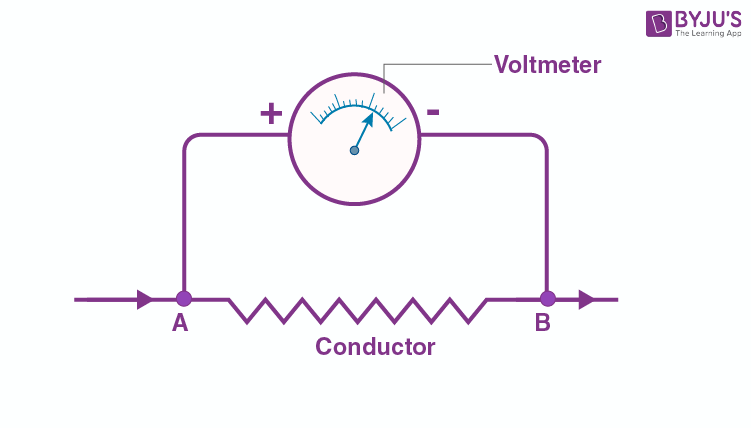e) The voltmeter has a high resistance because the voltmeter needs to take a negligible amount of current from an electric circuit.

Multiple Choice Questions

Q17. The work done in moving a unit charge across two points in an electric circuit is a measure of:

a) Current

b) Potential difference

c) Resistance

d) Power

The correct option is b) Potential difference.

Potential difference is the measure of the work done in moving a unit charge across two points in an electric circuit.

Q18. The device used for measuring potential difference is known as:

a) Potentiometer

b) Ammeter

c) Galvanometer

d) Voltmeter

The correct option is d) Voltmeter

Voltmeter is a device that is used for measuring the potential difference.

Q19. Which of the following units could be used to measure electric charge?

a) Ampere

b) Joule

c) Volt

d) Coulomb

The correct option d) Coulomb

Coulomb is the unit that is used for measuring electric charge.

Q20. The unit for measuring potential difference is:

a) Watt

b) Ohm

c) Volt

d) kWh

The correct option is c) Volt

Volt is the unit used for measuring the potential difference.

Q21. One coulomb charge is equivalent to the charge obtained in:

a) 2.6×1019 electrons

b) 6.2×1019 electrons

c) 2.65×1018 electrons

d) 6.25×1018 electrons

The correct option is d) 6.25×1018 electrons

There are 6.25×1018 electrons in one coulomb charge.

High Order Thinking Skills

Q22. Three 2V cells are connected in series and used as batteries in a circuit.

a) What is the p.d at the terminals of the battery?

b) How many joules of electric energy does 1C gain on passing through one cell and all three cells?

a) When three cells with 2 volts respectively are connected in series for the construction of the battery, then the total potential difference between the terminals of the battery will be 6V.

b) For one cell:

Given,

Potential difference = 2V

Charge moved = 1C

Work done = ?

We know that work done is given as:

Work done = potential difference × charge moved

= 2 × 1 = 2J

Therefore, work done to move a charge of 1C with the potential difference of 2V is 2J.

For three cells:

Given,

Potential difference = 6V

Change moved = 1C

Work done = ?

We know that the work done is given as:

Work done = Potential difference × charge moved

= 6 × 1 = 6J

Therefore, to move 1C charge with a potential difference of 6V, the work done is 6J.

Q23. The atoms of copper contain electrons, and the atoms of rubber also contain electrons. Then why does copper conduct electricity but rubber does not conduct electricity?

The atoms of copper contain electrons, and the atoms of rubber also contain electrons, but copper conducts electricity, while rubber doesn’t conduct electricity because copper has free electrons that are held loosely to each other by the nuclei of the atom. These freely available electrons are responsible for the conduction of electricity in copper.

Whereas in rubber, the electrons are under a strong influence of the nuclei of the atoms. There are not much free electrons available for the conduction of electricity in the rubber. Therefore, the rubber doesn’t conduct electricity.

Lakhmir Singh Physics Class 10 Solutions Page No.11

Q1. By what name is the physical quantity coulomb/second called?

Ampere is the name that is used for the physical quantity coulomb/second.

It is defined as the elementary charge e with a value of 1.602 × 10-19 is expressed in unit C which is equal to A.s

Q2. What is the flow of charge called?

The flow of charge is called an electric current.

Electric current is defined as the rate of flow of negative charges in the conductor. Or it can also be defined as the continuous flow of electrons in an electric circuit.

Q3. What actually travels through the wires when you switch on a light?

There is a flow of electrons in the wires when the light is switched on.

Q4. Which particles constitute the electric current in a metallic conductor?

Electrons are the particles that constitute the electric current in a metallic conductor.

Q5. a) In which direction does conventional current flow around a circuit?

b) In which direction do electrons flow?

a) The flow of conventional current takes place from the positive terminal of the batter to the negative terminal in the outer circuit.

b) The direction in which electrons flow is from the negative terminal to the positive terminal in the battery. The flow of electrons is usually in the opposite direction of the conventional current.

Q6. Which of the following equations shows the correct relationship between electrical units?

1A = 1C/s or 1C = 1A/s

The correct is 1A = 1C/s

Q7. What is the unit of electric current?

The unit of electric current is ampere.

Q8. a) How many milliamperes are there in 1 ampere?

b) How many microamperes are there in 1 ampere?

a) Following is the milliamperes that are there in 1 ampere:

1 amp = 103 milliamp

b) Following is the microamperes that are there in 1 ampere:

1 amp = 106 micro amp

Q9. Which of the two is connected in series: ammeter or voltmeter?

Out of the ammeter and voltmeter, the ammeter is connected in series.

Q10. Compare how an ammeter and a voltmeter are connected in a circuit.

In an electric circuit, the ammeter is connected in series while the voltmeter is connected in parallel.

Q11. What do the following symbols mean in circuit diagrams?a) The symbol above means variable resistance in the circuit diagram.

b) The symbol above means a closed plug key in the circuit diagram.

Q12. If 20C of charge passes a point in a circuit in 1s, what current is flowing?

Given,

Charge moved, Q = 20C

Time taken, t = 1s

To find,

Current, I = ?

We know that the current is calculated as follows:

I = Q/t

Substituting the values, we get

I = 20/1 = 20A

Therefore, 20A current is required to move a charge of 20C in 1s time.

Q13. A current of 4A flows around a circuit for 10s. How much charge flows past a point in the circuit in this time?

Given,

Current, I = 4A

Time taken, t = 10s

To find,

Charge moved, Q = ?

We know that,

I = Q/t

Substituting the values in the above equation, we get

4 = Q/10

Q = 4×10 = 40C

Therefore, 40C charge is moved in 10s when the current flowing is 4A.

Q14. What is the current in a circuit if the charge passing each point is 20C in 40s?

Given,

Charge moved, Q = 20C

Time taken, t = 40s

To find,

Current, I = ?

We know that,

I = Q/t

Substituting the values in the above equation, we get

I = 20/40 = 0.5A

Therefore, 0.5A is required for moving 20C charge in 40s.

Q15. Fill in the following blanks with suitable words:

a) A current is a flow of …….. for this to happen, there must be a ………

b) Current is measured in ……….. using an ………….placed in ……..in a circuit.

a) Electrons and closed

b) Amperes, ammeter, and series

Q16. a) Name a device which helps to maintain the potential difference across a conductor (say a bulb).

b) If a potential difference of 10V causes a current of 2A to flow for 1 minute, how much energy is transferred?

a) The potential difference across a conductor is maintained with the help of a cell or a battery.

b) Given,

Potential difference = 10V,

Current, I = 2A

Time taken, t = 60s

We know that:

I = Q/t

So, Q = I×t

Substituting the values, we get

Q = 2 × 60 = 120

Therefore, the charge moved is 120C

We also know that,

Work done = Potential difference × charge moved

= 120 × 10

=1200J

Therefore, the work done to move a charge of 120C is 1200J.

Q17. a) What is an electric current? What makes an electric current flow in a wire?

b) Define the unit of electric current.

a) An electric current is defined as the flow of electric charges through a conductor such that the potential difference between the two points in the conductor is responsible for the flow of electric current.

b) Ampere is the unit of electric current. It is defined as 1 coulomb of charge flowing through a cross-section of a conductor in 1 second such that the electric current flowing through the conductor is 1 ampere.

Q18. What is an ammeter? How is it connected in a circuit? Draw a diagram to illustrate your answer.

An ammeter is defined as a device that is used for measuring the flow of electric current. An ammeter is always connected in series with the electric current through which the current needs to be measured. Following is the illustration of the connection of the ammeter in series with the electric circuit: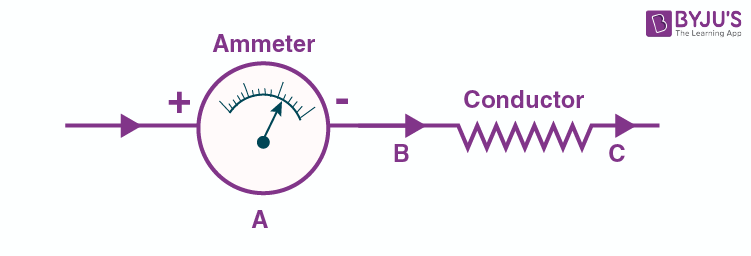Q19. a) Write down the formula which relates electric charge, time, and electric current.

b) A radio set draws a current of 0.36A for 15 minutes. Calculate the amount of electric charge that flows through the circuit.

a) The relation between electric charge, time, and electric current is given as:

Current = (charge moved)/(time)

While work done = potential difference × charge moved

Therefore, above is the relation between electric, time, and electric current

b) Given,

Current, I = 0.36A

Time taken, t = 15 min = 900s

We know that,

Q = I × t

= 0.36 × 900

= 324C

Therefore, 324C charge flows through the circuit.

Q20. Why should the resistance of:

a) An ammeter be very small?

b) A voltmeter be very large?

a) The resistance of an ammeter should be very small because the value of the current flowing through the circuit remains unchanged.

b) The resistance of a voltmeter should be very large because the current that flows through the voltmeter should be of negligible value.

Q21. Draw circuit symbols for

a) fixed resistance

b) variable resistance

c) a cell

d) a battery of three cells

e) an open switch

f) a closed switch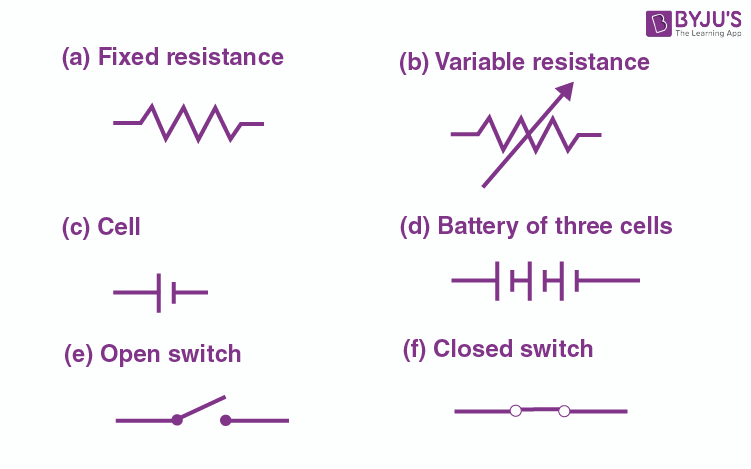Q22. What is a circuit diagram? Draw the labelled diagram of an electric circuit comprising of a cell, a resistor, an ammeter, a voltmeter, and a closed switch or closed plug key. Which of the two has a large resistance: an ammeter or a voltmeter?

A circuit diagram is defined as a diagram which is used for indicating the different components in an electric circuit and those that are connected with the help of electric symbols.

Also, a voltmeter will have a high resistance than an ammeter.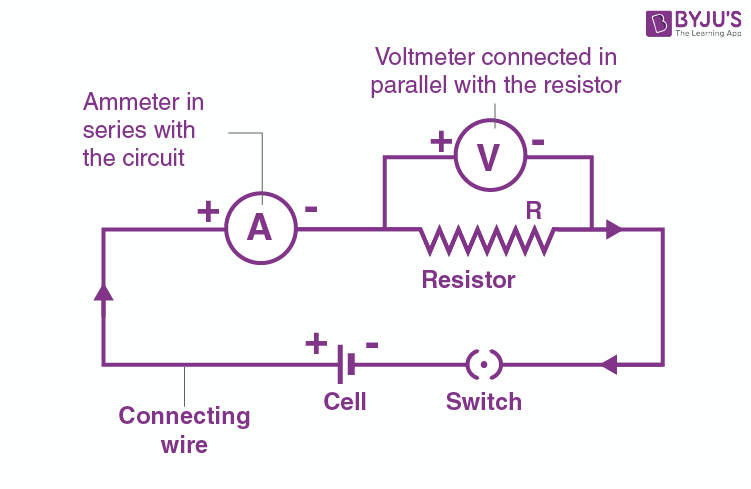Q23. If the charge on an electron is 1.6 × 10-19 coulombs, how many electrons should pass through a conductor in 1 second to constitute 1 ampere current?

Given,

Charge moved = 1.6 × 10-19 coulombs

Current, I = 1A

Time taken, t = 1s

We know that,

I = Q/t

1 = Q/1

Q = 1C

We also know that when the charge is 1.6 × 10-19 coulombs, the number of electrons present is 1

Therefore, when a charge of 1 coulomb is present, the number of electrons is given as:

(1)/( 1.6 × 10-19) = 0.625 × 10-19 = 6.25 × 1018

Q24. The potential difference across a lamp is 12V. How many joules of electrical energy is changed into heat and light when:

a) a charge of 1C passes through it?

b) a charge of 5C passes through it?

c) a current of 2A flows through it for 10s?

Given,

Potential difference = 12V

a) Potential difference = (work done)/(charge moved)

Work done = Potential difference × charge moved

= 12 × 1 = 12J

Therefore, 12J of electrical energy is converted into heat and light.

b) Work done = potential difference × charge moved

= 12 × 5 = 60J

Therefore, 60J of electrical energy is converted into heat and light.

c) I = Q/t

Q = I × t

= 2 × 10 = 20C

Work done = potential difference × charge moved

= 12 × 20 = 240J

Therefore, 240J of electrical energy is converted into heat and light.

Lakhmir Singh Physics Class 10 Solutions Page No.12

Q25. In 10s, a charge of 25C leaves a battery, and 200J of energy is delivered to an outside circuit as a result.

a) What is the potential difference across the battery?

b) What current flows from the battery?

Given,

Time taken, t = 10s

Charge moved, Q = 25C

Energy delivered is work done = 200J

a) Potential difference = (work done)/(charge moved) = (200)/(25) = 8V

b) I = Q/t = 25/10 = 2.5A

Q26. a) Define electric current. What is the SI unit of electric current?

b) One coulomb of charge flows through any cross-section of a conductor in 1 second. What is the current flowing through the conductor?

c) Which instrument is used to measure electric current? How should it be connected in a circuit?

d) What is the conventional direction of the flow of electric current? How does it differ from the direction of the flow of electrons?

e) A flash of lightning carries 10C of charge, which flows for 0.01s. What is the current? If the voltage is 10MV, what is the energy?

a) The flow of electric charges or electrons in a conductor, such as a metal wire, is known as electric current. Ampere is the SI unit of electric charges.

b) The current flowing through the conductor when one coulomb of charge flows through any cross-section of a conductor in 1 second is 1 ampere.

c) For the measurement of electric current, an ammeter is used, which is connected in series in the circuit.

d) The direction of the conventional direction of flow of electric current takes place from the positive terminal of a battery to the negative terminal across the outer circuit. It is different from the direction of the flow of electrons because the electrons flow from the negative terminal to the positive terminal.

e) Given,

Charge moved, Q = 10C

Time taken, t = 0.01s

We know that,

Current, I = Q/t = 10/0.01 = 1000A

Potential difference = Work done/ charge moved

Work done = potential difference × charge moved = 10 × 106 × 10 = 100 × 106 = 100MJ

Energy = work done = 100MJ

Multiple Choice Questions

Q27. The other name of potential difference is

a) Ampereage

b) Waltage

c) Voltage

d) Potential energy

The other name of potential difference is c) Voltage

Q28. Which statement/statements is/are correct?

a) An ammeter is connected in series in a circuit and a voltmeter is connected in parallel

b) An ammeter has a high resistance

c) A voltage has a low resistance

The correct statement is a) An ammeter is connected in series in a circuit, and a voltmeter is connected in parallel

Q29. Which unit could be used to measure current?

a) Watt

b) Coulomb

c) Volt

d) Ampere

The correct option that could be used to measure the current unit is d) Ampere

Q30. If the current through a flood lamp is 5A, what charge passes in 10 seconds?

a) 0.5C

b) 2C

c) 5C

d) 50C

Given,

Current, I = 5A

Time taken, t = 10s

Charge moved, Q = ?

I = Q/t

Q = I × t

Q = 50C

Therefore, the correct option is d) 50C

Q31. If the amount of electric charge passing through a conductor in 10 minutes is 300C, the current flowing is:

a) 30A

b) 0.3A

c) 0.5A

d) 5A

Given,

Charge moved = 300C

Time taken = 10 mins

Current = ?

I = Q/t

Substituting, we get I = 0.5A

Therefore, the correct option is c) 0.5A

High Order Thinking Skills

Q32. A student made an electric circuit shown here to measure the current through two lamps.

a) Are the lamps in series or parallel?

b) The student has made a mistake in this circuit. What is the mistake?

c) Draw a circuit diagram to show the correct way to connect the circuit. Use the proper circuit symbols in your diagram.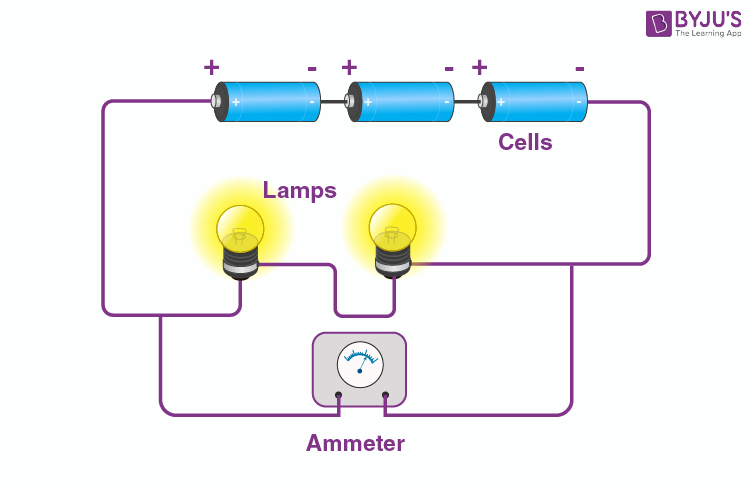a) The lamps are arranged in series.

b) The mistake made by the student is that the ammeter is connected in parallel. It should be connected in series.

c)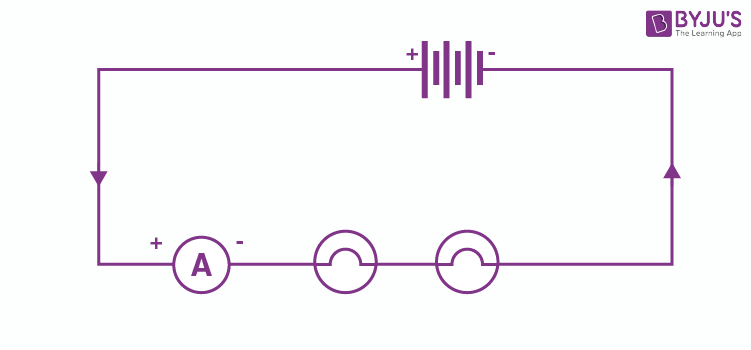Q33. Draw a circuit diagram to show how 3 bulbs can be lit from a battery so that 2 bulbs are controlled by the same switch while the third bulb has its own switch.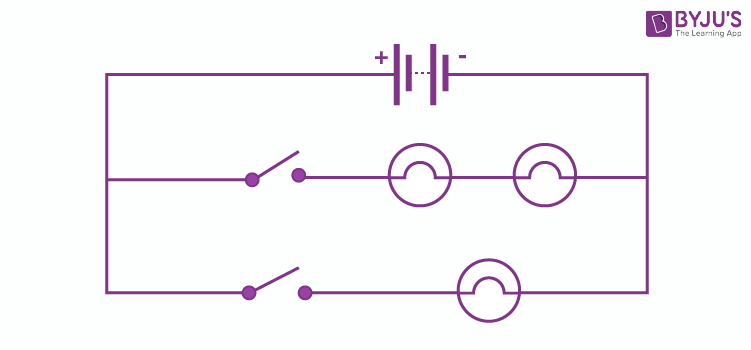Q34. An electric heater is connected to the 230V mains supply. A current of 8A flows through the heater.

a) How much charge flows around the circuit each second?

b) How much energy is transferred to the heater each second?

Given,

Potential difference = 230V

Current, I = 8A

a) I = Q/t

8 = Q/1

Q = 8 × 1 = 8C

Therefore, the charge flows around the circuit for every second is 8C.

b) We know that energy transferred = work done

Potential difference = work done/charge moved

230 = work done/8

Therefore, work done = 230 × 8 = 1840J

Q35. How many electrons are flowing per second past a point in a circuit in which there is a current of 5A?

Given,

Current, I = 5A

Time taken, t = 1s

We know that,

I = Q/t

Q = I × t = 5 × 1 = 5C

Number of electrons present in 1.6 × 10-19C = 1

Number of electrons present in 5C = (5)/( 1.6 × 10-19) = 31.25 × 1018 C

Page No: 18

Q1. Name the law which relates the current in a conductor to the potential difference across its ends.

The law that relates the current in a conductor to the potential difference across its ends is Ohm’s law.

Q2. Name the unit of electrical resistance and give its symbol.

The unit of electrical resistance is Ohm. Following is the symbol of ohm: Ω

Q3. Name the physical quantity whose unit is “ohm”.

The physical quantity whose unit is “ohm” is electric resistance.

Q4. What is the general name of the substances having infinitely high electrical resistance?

Insulators are substances that have infinitely high electrical resistance.

Q5. Keeping the resistance constant, the potential difference applied across the ends of a component is halved. By how much does the current change?

Given,

Resistance = constant

We know that V = IR

When resistance, R is constant, V is proportional to I

Therefore, when V is halved, I also becomes half.

Q6. State the factors on which the strength of the electric current flowing in a given conductor depends.

The following are the factors on which the strength of the electric current flowing in a given conductor depends:

a) Voltage (or potential difference) across the ends of the conductor

b) Resistance offered by the conductor

Q7. Which has less electrical resistance: a thin wire or a thick wire of the same length and the same material?

A thick wire with the same length and same material as that of a thin wire will have less electrical resistance because the number of electrons present in the thick wire is more than the thin wire.

Q8. Keeping the potential difference constant, the resistance of a circuit is halved. By how much does the current change?

Given,

Potential difference = constant

Also, we know that,

V = IR

I = V/R

Given that V is constant, therefore, I will be proportional to 1/R.

Therefore, when R is halved, I also becomes double.

Q9. A potential difference of 20V is applied across the ends of a resistance of 5 ohms. What current will flow in the resistance?

Given,

Potential difference, V = 20V

Resistance, R = 5 Ohms

Current, I = ?

We know that,

V = IR

20 = I × 5

I = 20/5 = 4A

Q10. A resistance of 20 ohms has a current of 2 amperes flowing in it. What potential difference is there between its ends?

Given,

Resistance, R = 20 Ohms

Current, I = 2 amp

We know that,

V = IR

Therefore, V = 2 × 20 = 40V

Q11. A current of 5 amperes flows through a wire whose ends are at a potential difference of 3 volts. Calculate the resistance of the wire.

Given,

Current, I = 5A

Potential difference, V = 3V

We know that,

V = IR

Therefore,

3 = 5 × R

R = 3/5 = 0.6 Ohms

Q12. Fill in the following blanks with a suitable word:

Ohm’s law states a relation between potential difference and ……

Ohm’s law states a relation between potential difference and current.

Q13. Distinguish between good conductors, resistors, and insulators. Name two conductors, two resistors, and two insulators.

The difference between good conductors, resistors, and insulators are as follows:

Materials that have less or low electrical resistance are known as good conductors.

Copper and iron are two examples of good conductors.

The materials that have comparatively high resistance are known as resistors.

Manganin and nichrome are two examples of resistors.

Materials that have infinitely high resistance are known as insulators.

Wood and rubber are two examples of insulators.

Q14. Classify the following into good conductors, resistors, and insulators:

Rubber, mercury, nichrome, polyethene, aluminium, wood, manganin, bakelite, iron, paper, thermocol, metal coin

Conductor: mercury, aluminium, iron, metal coin

Resistor: manganin, nichrome

Insulator: rubber, polythene, wood, bakelite, paper, thermocol

Lakhmir Singh Physics Class 10 Solutions Page No:19

Q15. What is Ohm’s law? Explain how it is used to define the unit of resistance.

Ohm’s law helps in understanding the relation between current, I and potential difference, V. The law states that at a constant temperature, the current flowing through a conductor is directly proportional to the potential difference across its ends. The mathematical representation of the law is:

Let I be the current flowing through a conductor, and V be the potential difference across the ends of the conductor. Then from Ohm’s law, we know that

I ∝ V

V ∝ I

V = RI

R = v/I

Where,

R is the resistance offered by the conductor, which is constant. The unit of resistance is ohm.

If V = 1 volt and I = 1mp, then R = 1/1 = 1ohm.

Therefore, 1 ohm is defined as the resistance of a conductor such that when the potential difference of 1 volt is applied to its ends, a current of 1 amp flows through it.

Q16. a) What is meant by the “resistance of a conductor”? Write the relation between resistance, potential difference, and current.

b) When a 12V battery is connected across an unknown resistor, there is a current of 2.5mA in the circuit. Calculate the value of the resistance of the resistor.

a) Resistance of a conductor is the property of a conductor. This property helps the conductor in opposing the flow of current through it.

Work done = potential difference × charge moved

b) Given,

Voltage, V = 12 volt

Current, I = 2.5 × 10-3A

We know that,

V = IR

R = V/I

R = 12/2.5 × 10-3

R = 4.8 × 103ohm = 4800 ohm

Q17. a) Define the unit of resistance.

b) What happens to the resistance as the conductor is made thinner?

c) Keeping the potential difference constant, the resistance of a circuit is doubled. By how much does the current change?

a) The unit of resistance is ohm. 1 ohm is defined as the resistance offered by the conductor such that when 1 volt potential difference is applied, then 1 ampere current flows through the conductor.

b) As the conductor is made thinner, the resistance of the conductor increases.

c) By keeping the potential difference constant and the resistance is doubled, then

V = IR

I = V/R

Then, I ∝ 1/R

Therefore, when R is doubled, I becomes half.

Q18. a) Why do electricians wear rubber hand gloves while working with electricity?

b) What potential difference is needed to send a current of 6A through an electrical appliance having a resistance of 40Ω?

a) We know that rubber is a good insulator or a bad conductor of electricity. This is the reason why electricians wear rubber hand gloves while working with electricity.

b) Given,

Current, I = 6A

Resistance, R = 40Ω

We know that,

V = IR

V = 6 × 40 = 240V

Q19. An electric circuit consisting of a 0.5m long nichrome wire XT, an ammeter, a voltmeter, four cells of 1.5V each and a plug key was set up.

a) Draw a diagram of this electric circuit to study the relation between the potential difference maintained between the points ‘X’ and ‘Y’ and the electric current flowing through XY.

b) Following graph was plotted between V and I values: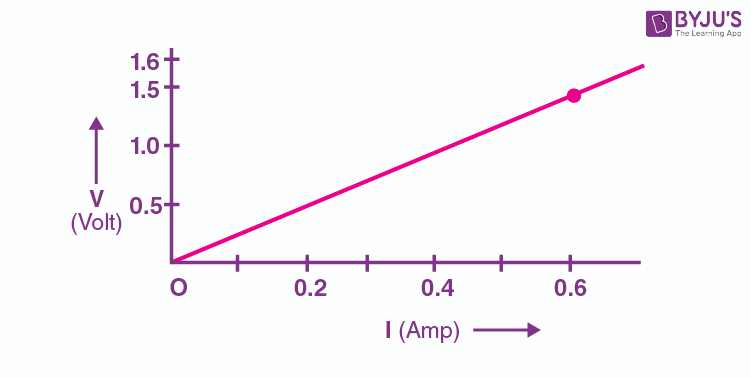What would be the values of 1/V ratios when the potential difference is 0.8V, 1.2V, and 1.6V, respectively? What conclusion do you draw from these values?

c) What is the resistance of the wire?

a)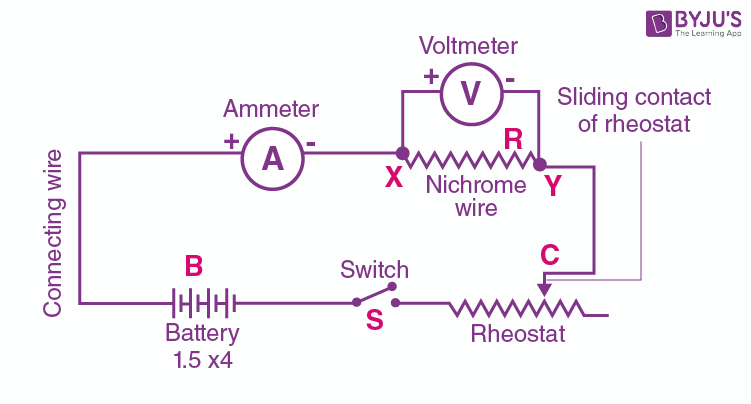b) As we see that the graph is a straight line passing through the origin, we can say that the current is directly proportional to the potential difference. Therefore, the ratio V/I remains constant.

From graph,

V = 1.5 volt

I = 0.6 amp

Therefore, V/I = 1.5/0.6 = 2.5Ω

For the potential difference of 0.8V, 1,2V, and 1.6V the value of V/I ratio remains the same which is 2.5 ohm.

c) The resistance of the wire and the ratio of potential difference and the current passing through the wire are equal.

R = V/I = 2.5 Ω

Q20. a) What the ratio of potential difference and current is known as?

b) The values of potential difference V applied across a resistor and the corresponding values of current I flowing in the resistor are given below:

 Potential difference, V in volts 2.5 5 10 15 20 25 Current, I in amperes 0.1 0.2 0.4 0.6 0.8 1

Plot a graph between V and I and calculate the resistance of the resistor.

c) Name the law which is illustrated by the above V-I graph

d) Write down the formula which states the relation between potential difference, current, and resistance

e) The potential difference between the terminals of an electric iron is 240V, and the current is 5.0A. What is the resistance of the electric iron?

a) The ratio of potential difference and the current is known as resistance.

c) The law illustrated by the V-I graph is Ohm’s law.

d) Following is the formula that states the relation between potential difference, current, and resistance:

Potential difference = Current × Resistance

e) Given,

Voltage, V = 240 volt

Current, I = 5 amp

We know that

V = IR

240 = 5 × R

R = 240/5 = 48 ohm

Multiple Choice Questions

Q21. The potential difference across a 3Ω resistor is 6V. The current flowing in the resistor will be:

a) ½ A

b) 1A

c) 2A

d) 6A

The correct option is c) 2A.

Q22. A car headlight bulb working on a 12V car battery draws a current of 0.5A. The resistance of the light bulb is:

a) 0.5Ω

b) 6 Ω

c) 12 Ω

d) 24 Ω

The correct option is d) 24 Ω

Q23. An electrical appliance has a resistance of 25 Ω. When this electrical appliance is connected to a 230 Ω supply line, the current passing through it will be:

a) 0.92A

b) 2.9A

c) 9.2A

d) 92A

The correct option is c) 9.2A

Q24. When a 4 Ω resistor is connected across the terminals of a 12V battery, the number of coulombs passing through the resistor per second is:

a) 0.3

b) 3

c) 4

d) 12

The correct option is b) 3

Q25. Ohm’s law gives a relation between:

a) Current and resistance

b) Resistance and potential difference

c) Potential difference and electric charge

d) Current and potential difference

The correct option that gives the relation in Ohm’s law is d) current and potential difference.

Q26. The unit of electrical resistance is:

a) Ampere

b) Volt

c) Coulomb

d) Ohm

The correct option for the unit of electrical resistance is d) Ohm.

Q27. The substance having infinitely high electrical resistance is called:

a) Conductor

b) Resistor

c) Superconductor

d) Insulator

The correct option for the substance with infinitely high electrical resistance is called d) insulator.

Q28. Keeping the potential difference constant, the resistance of a circuit is doubled. The current will become:

a) Double

b) Half

c) One-fourth

d) Four times

When the potential difference is constant and the resistance of a circuit is doubled, the current becomes half.

Therefore, the correct option is b) half.

Q29. Keeping the potential difference constant, the resistance of a circuit is halved. The current will become:

a) One-fourth

b) Four times

c) Half

d) Double

When the potential difference is constant and the resistance of a circuit is halved, then the current will be doubled.

Therefore, the correct option is d) doubled.

High Order Thinking Skills

Q30. An electric room heater draws a current of 2.4A from the 120V supply line. What current will this room heater draw when connected to 240V supply line?

First case:

Given,

Current, I = 2.4amp

Voltage, V = 120V

We know that,

V = IR

120 = 2.4 × R

R = 120/2.4 = 50 Ohm

Second case:

Given,

Voltage, V = 240 volt

Resistance, r = 50 Ohm

We know that,

V = IR

240 = I × 50

I = 4.8 amp

Q31. Name the electrical property of a material whose symbol is “omega”.

The electrical property resistance of the material has the symbol “omega”.

Q32. The graph between V and I for a conductor is a straight line passing through the origin.

a) Which law is illustrated by such a graph?

b) What should remain constant in a statement of this law?

a) Ohm’s law is illustrated by the V-I graph in which the straight line passes through the origin.

b) For Ohm’s law to hold good in the given situation, temperature must remain constant.

Q33. A potential difference of 10V is needed to make a current of 0.02A flow through a wire. What is the potential difference needed to make a current of 250mA flow through the same wire?

First case,

Given,

Current, I = 0.02A

Voltage, V = 10 volt

We know that,

V = IR

10 = 0.02 × R

R = 10/0.02 = 500 Ohms

Second case,

Current, I = 250 × 10-3 A

Resistance, R = 500 Ohm

V = IR

V = 250 × 10-3 × 500 = 125 volt

Q34. A current of 200mA flows through a 4kΩ resistor. What is the potential difference across the resistor?

Given,

Current, I = 200mA = 0.2A

Resistance, R = 4kΩ = 4 × 103 Ohm = 4000 Ohm

We know that,

V = IR

V = 0.2 × 4000

V = 800 volt

Page No: 26

Q1. What happens to the resistance as the conductor is made thicker?

As the thickness of the conductor is increased, the resistance of the conductor decreases. This is because:

R = (ρl)/(A)

Where,

R is the resistance

l is the length of the conductor

A is the area of cross-section of the conductor

And ρ is the resistivity

Since the area is directly proportional to the radius of the wire, an increase in cross-sectional area or thickness will result in a decrease in resistance and current flow will be more.

Q2. If the length of a wire is doubled by taking more of the wire, what happens to its resistance?

The resistance of the wire becomes double when the length of the wire is doubled. This is because length is directly proportional to the resistance while the area of the cross-section is inversely proportional to the resistance.

Also, we know that R = (ρl)/(A)

Therefore, if l becomes double, then R becomes 2R.

Q3. On what factors does the resistance of a conductor depend?

The following are the factors on which the resistance of a conductor depends:

a) Length of the conductor

b) Area of cross-section of the conductor

c) Temperature of the conductor

d) Nature of the material used in the construction of the conductor

Q4. Name the material which is the best conductor of electricity.

Silver metal is the best conductor of electricity. This is because the electrons of silver metal are available for free movement when compared to the other metals.

Q5. Which among iron and mercury is a better conductor of electricity?

Among iron and mercury, iron is a better conductor of electricity. The resistance of iron is less than mercury, and we know that resistance is inversely proportional to the current. Therefore, iron is better as a conductor of electricity when compared to mercury.

Q6. Why are copper and aluminium wires usually used for electricity transmission?

Copper and aluminium wires are used for electricity transmission because they have low resistivities.

Q7. Name the material which is used for making the heating element of an electric iron.

Nichrome is the material which is used for making the heating element of an electric iron.

Q8. What is nichrome? State its one use.

Nichrome is an alloy material obtained from nickel, chromium, manganese, and iron. The resistivity of nichrome is 60 times more than that of copper. It is used in the making of the elements that are used for heating in most of the electrical heating appliances.

Q9. Give two reasons why nichrome alloy is used for making the heating elements of electrical appliances.

Following are the two reasons why nichrome alloy is used for making the heating elements of electrical appliances:

a) The resistivity of nichrome is very high.

b) Nichrome doesn’t undergo oxidation at high temperatures.

Q10. Why are the coils of electric iron and electric toasters made of an alloy rather than a pure metal?

The coils of an electric iron and electric toasters are made of an alloy rather than a pure metal because:

a) The resistivity of an alloy is higher when compared to the pure metal

b) Alloys don’t undergo oxidation at high temperatures

Q11. Which has more resistance:

a) A long piece of nichrome wire or a short one?

b) A thick piece of nichrome wire or a thin piece?

The following has more resistance:

a) A long piece of nichrome wire

b) A thin piece of nichrome wire

Q12. a) How does the resistance of a pure metal change if its temperature decreases?

b) Hoes does the presence of impurities in a metal affect its resistance?

a) As the temperature decreases, the resistance of the material also decreases.

b) Presence of impurities in a metal increases the resistance of the material.

Q13. Fill in the following blanks with suitable words:

Resistance is measured in ………… the resistance of a wire increases as the length ………….. as the temperature ……… and as the cross-sectional area ……..

Ohm, increases, increases, and decreases.

Q14. a) What do you understand by the “resistivity” of a substance?

b) A wire is 1.0m long, 0.2 mm in diameter and has a resistance of 10Ω. Calculate the resistivity of its material.

a) Resistivity is defined as the characteristic property of a substance which is dependent on the nature of the substance and the temperature of the substance used in the conductor. It is numerically equal to the resistance between the opposite faces of a cube with sides 1m.

b) Given,

l = 1m

r = d/2 = 0.2/2 = 0.1mm = 0.0001m

R = 10ohm

We know that,

R = ρl/A

ρ = RA/l

= 31.4×10-8ohm

Q15. a) Write down an expression for the resistance of a metallic wire in terms of resistivity.

b) What will be the resistance of a metal wire of length 2 metres and area of cross-section 1.55×10-6m2, if the resistivity of the metal is 2.8×10-8Ωm?

a) Following is the expression for the resistance of a metallic wire in terms of the resistivity:

R = ρl/A

Where,

R is the resistance of the wire

ρ is the resistivity of the wire

A is the cross-sectional area of the wire

l is the length of the wire

b) Given,

l = 2m

A = 1.55×10-6m2

ρ = 2.8×10-8Ωm

We know that,

R = ρl/A

= 0.036 ohm

Q16. a) Give two examples of substances which are good conductors of electricity. Why do you think they are good conductors of electricity?

b) Calculate the resistance of a copper wire 1.0km long and 0.50mm diameter if the resistivity of copper is 1.7×10-8Ωm.

a) Two examples of substances that are good conductors of electricity are silver and copper. These two metals have free electrons that are available for conduction.

b) Given,

l = 1km = 1000m

r = d/2

= 0.5/2mm

= 0.25mm

= 0.25×10-3m

ρ = 1.7×10-8Ωm

We know that,

R = ρl/A

R = 86.6Ω

Q17. Will current flow more easily through a thick wire or a thin wire of the same material when connected to the same source? Give reasons for your answer.

The flow of current will be easy in a thick wire than the thin wire because the resistance of the thick wire will be lesser than the thin wire.

Q18. How does the resistance of a conductor depend on:

a) length of the conductor

b) area of cross-section of the conductor

c) temperature of the conductor

a) Resistance of a conductor is dependent on the length of the conductor. As the length of the material increases, the resistance of the material decreases and vice versa.

b) Resistance of a conductor is dependent on the area of cross-section of the conductor. As the cross-section area increases, the resistance of the material decreases and vice versa.

c) As the temperature increases, the resistance of the material also increases.

Q19. a) Give one example to show how the resistance depends on the nature of the material of the conductor

b) Calculate the resistance of an aluminium cable of length 10km and diameter 2.0mm if the resistivity of aluminium is 2.7×10-8Ωm.

a) Let us consider two wires with the same length and diameter. Let these two wires be of copper metal and nichrome alloy. We know that the resistance of nichrome wire is 60 times more than that of copper wire. Therefore, it is understood that the resistance depends on the nature of the material used in the conductor.

b) Given,

l = 10km = 10000m

d = 2mm

r = 1mm = 10-3m

ρ = 2.7×10-8Ωm

We know that,

R = ρl/A

Substituting the values we get,

R = 86Ω

Q20. What would be the effect on the resistance of a metal wire of:

a) increasing its length

b) increasing its diameter

c) increasing its temperature

The following are the effects on the resistance of a metal wire when:

a) As the length is increased, the resistance will increase

b) As the diameter is increased, the resistance will decrease

c) As the temperature is increased, the resistance will increase

Q21. How does the resistance of a wire vary with its:

a) area of cross-section?

b) diameter?

a) When the resistance of a wire is varied, the area of cross-section increases, thereby reducing the resistance

b) When the resistance of a wire is varied, the diameter of the material is increased, thereby reducing the resistance

Q22. How does the resistance of a wire change when:

a) its length is tripled?

b) its diameter is tripled?

c) its material is changed to one whose resistivity is three times?

We know that,

R = ρl/A

a) l becomes 3l

R’ = ρ3l/A

= 3R

Therefore, resistance gets tripled

b) d becomes 3d

R = ρl/A

R = ρl/πr2

= ρl/π(d/2)2

R’ = R/9

Therefore, resistance becomes 1/9th

c) ρ = 3ρ

R’ = 3ρl/A

= 3R

Therefore, resistance becomes 3 times.

Q23. Calculate the area of cross-section of a wire if its length is 1.0m, its resistance is 23Ω, and the resistivity of the material of the wire is 1.84×10-6Ωm.

Given,

l = 1.0m

R = 23 ohm

ρ = 1.84×10-6Ωm

We have,

R = ρl/A

23 = 1.84×10-6 × 1/A

A = 8×10-8 m2

Q24. a) Define resistivity. Write an expression for the resistivity of a substance. Give the meaning of each symbol.

b) State the SI unit of resistivity.

c) Distinguish between resistance and resistivity.

d) Name two factors on which the resistivity of a substance depends and two factors on which it does not depend.

e) The resistance of a metal wire of length 1m is 26Ω at 20C. if the diameter of the wire is 0.3mn, what will be the resistivity of the metal at that temperature?

a) Resistivity is defined as:

ρ = R×A/l

where,

R is the resistance of the conductor

A is the cross-sectional area of the conductor

l is the length of the conductor

b) The SI unit of resistivity is Ohm-meter

c) Following are the differences between resistance and resistivity:

 Resistance Resistivity Resistance is the property of the conductor Resistivity is the property of the material used in the conductor In a conductor, resistance is defined as the opposing force to the flow of electric current Resistivity is the opposing force of the flow of electric current in a rod such that the length of the rod is 1m and the cross-sectional area of the rod is 1m2 Parameters such as length, nature of the material used in a conductor, and thickness of the conductor determine the resistance Parameters such as length and thickness do not determine the resistivity of the conductor

d) Nature of the material and the temperature of the material determine the resistivity of a substance. Resistivity is independent of the length and thickness of the conductor.

e) Given,

l = 1m

R = 26Ω

r = d/2

= 0.3/2

= 0.15mm

= 0.15×10-3m

We know that,

ρ = R×A/l

= 1.83×10-6Ωm

Multiple Choice Questions

Q25. The resistance of a wire of length 300m and cross-section area 1.0mm2 made of material of resistivity 1.0×10-7 Ωm is:

a) 2Ω

b) 3Ω

c) 20Ω

d) 30Ω

The correct option is d) 30Ω

We know that

R = ρl/A

Substituting the values in the above equation, we get 30Ω.

Q26. When the diameter of a wire is doubled, its resistance becomes:

a) Double

b) Four times

c) One-half

d) One-fourth

The correct option is d) one-fourth

We know that

R = ρl/A

Therefore, when the diameter of the wire is doubled, the resistance becomes one-fourth of the actual value.

Q27. If the resistance of a certain copper wire is 1Ω, then the resistance of a similar nichrome wire will be about:

a) 25 Ω

b) 30 Ω

c) 60 Ω

d) 45 Ω

The correct option is c) 60 Ω.

The resistance of nichrome is 60 Ω when the resistance of a copper wire is 1 Ω.

Q28. If the diameter of a resistance wire is halved, then its resistance becomes:

a) Four times

b) Half

c) One-fourth

d) Two times

The correct option is a) four times.

When the diameter of a resistance wire is halved, the resistance becomes four times.

Q29. The resistivity of a certain material is 0.6 Ωm. The material is most likely to be:

a) An insulator

b) A superconductor

c) A conductor

d) A semiconductor

The correct option is d) A semiconductor

Q30. When the area of cross-section of a conductor is doubled, its resistance becomes:

a) Double

b) Half

c) Four times

d) One-fourth

The correct option is b) half

Q31. The resistivity of copper metal depends on only one of the following factors. This factor is:

a) Length

b) Thickness

c) Temperature

d) Area of cross-section

The correct option is c) Temperature

Q32. If the area of cross-section of a resistance wire is halved, then its resistance becomes:

a) One-half

b) 2 times

c) One-fourth

d) 4 times

The correct option is b) 2 times.

High Order Thinking Skills

Q33. A piece of wire of resistance 20Ω is drawn out so that its length is increased to twice its original length. Calculate the resistance of the wire in the new situation.

We know that,

R = ρl/A

Given that,

l’ = 2l

A’ = A/2

And ρ’ = ρ (as the material of the wire is the same)

Therefore,

R’ = ρ’l’/A’

= ρ2l/(A/2)

= 4ρl/A

= 4R

Therefore, R’ = 4×20 = 80Ω

Q34. The electrical resistivities of three materials P, Q, and R are given below:

P = 2.3×103 Ωm

Q = 2.63×10-8 Ωm

R = 1.0×1015 Ωm

Which material will you use for making it?

a) Electric wires

b) Handle for soldering iron

c) Solar cells

a) For making the electric wires, Q = 2.63×10-8 Ωm can be used as this material has a very low resistivity

b) For making the handle for the soldering iron, R = 1.0×1015 Ωm can be used as this has a very high resistivity

c) For making the solar cells, P = 2.3×103 Ωm can be used as this material is a semiconductor

Q35. The electrical resistivities of four materials, A, B, C, and D, are given below:

A = 110×10-8 Ωm

B = 1.0×1010 Ωm

C = 10.0×10-8 Ωm

D = 2.3×103 Ωm

Which material is:

a) Good conductor

b) Resistor

c) Insulator

d) Semiconductor

a) Good conductor is C = 10.0×10-8 Ωm

b) Resistor is A = 110×10-8 Ωm

c) Insulator is B = 1.0×1010 Ωm

d) Semiconductor is D = 2.3×103 Ωm

Q36. The electrical resistivities of five substances, A, B, C, S, and E, are given below:

A =  5.20×10-8 Ωm

B =  110×10-8 Ωm

C =  2.60×10-8 Ωm

D =  10.0×10-8 Ωm

E =  1.70×10-8 Ωm

a) Which substance is the best conductor of electricity? Why?

b) Which one is a better conductor: A or C? Why?

c) Which substance would you advise to be used for making heating elements of electric irons? Why?

d) Which two substances should be used for making electric wires? Why?

a) E is the best conductor of electricity because it has the least resistivity

b) C is a better conductor between A and C because the resistivity of C is lesser than that of A

c) B can be used in the making of the heating elements of an electric iron because the resistivity of B is the highest

d) C and E are the two substances that should be used for making electric wires because they have low electrical resistivities

Page No: 37

Q1. Give the law of combination of resistances in series.

The law of combination of resistances in series states that the combined resistance of any number of resistance that are connected in series is equal to the individual resistances.

Q2. If five resistances each of value 0.2 ohm, are connected in series, what will be the resultant resistance?

According to the law of combination of resistances in series,

R = R1 + R2 + R3 + R4 + R5

R = 0.2 + 0.2 + 0.2 + 0.2 + 0.2 = 1 ohm

Q3. State the law of combination of resistance in parallel.

According to the law of combination of resistance in parallel, the reciprocal of the combined resistance of a number of resistances connected in parallel is equal to the sum of the reciprocals of all the individual resistances.

Q4. If 3 resistances of 3 ohm each are connected in parallel, what will be their total resistance?

R1 = R2 = R3 = 3Ω

1/R = 1/R1 + 1/R2 + 1/R3

= 1/3 + 1/3 + 1/3

= 3/3

= 1

Therefore, R = 1Ω

Q5. How should the two resistances of 2 ohms each be connected so as to produce an equivalent resistance of 1 ohm?

To produce an equivalent resistance of 1 ohm, the two resistances of 2 ohms must be connected in parallel.

Q6. Two resistances X and Y are connected turn by turn: a) in parallel b) in series. In which case the resultant resistance will be less than either of the individual resistances?

When the two resistance X and Y are connected in parallel, the resultant resistance will be less than either of the individual resistances.

Q7. What possible values of resultant resistance can one get by combining two resistances, one of value 2 ohm and the other 6 ohm?

R1 = 2 Ohm

R2 = 6 Ohm

Let case 1 be a parallel combination

1/R = 1/R1 + 1/R2

1/R = ½ + 1/6 = 4/6

R = 6/4 = 1.5 ohm

Let case 2 be a series combination

R = R1 + R2

= 2+6 = 8 ohm

Q8. Show how you would connect two 4 ohm resistors to produce a combined resistance of

a) 2 ohms

b) 8 ohms

a) By connecting in parallel, then

1/R = ¼ + ¼  = 2/4 = ½

Therefore, R = 2 ohm

b) By connecting in series, then

R = 4 + 4 = 8

Therefore, R = 8 ohm

Q9. Which of the following resistor arrangement, A or B, has the lower combined resistance?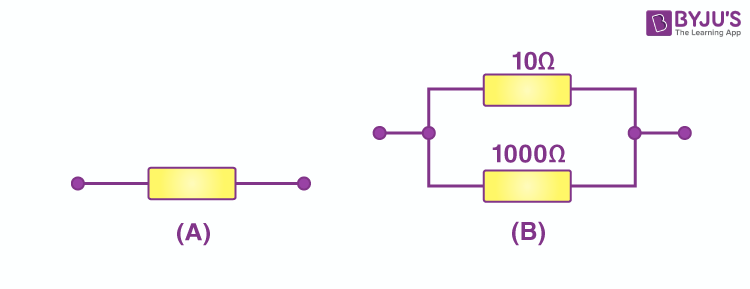When the resistance arrangement from A is combined with the resistance arrangement B, following is calculated:

1/R = 1/10 + 1/1000

= (100+1)/1000

R = 1000/101

R = 9.9 Ohm

Therefore, arrangement B has a lower combined resistance.

Q10. A wire that has resistance R is cut into two equal pieces. The two parts are joined in parallel. What is the resistance of the combination?

Given that the resistance of each part is: R/2

Let resultant resistance be R’

1/R’ = 2/R + 2/R

R’ = R/4

Q11. Calculate the combined resistance in each case: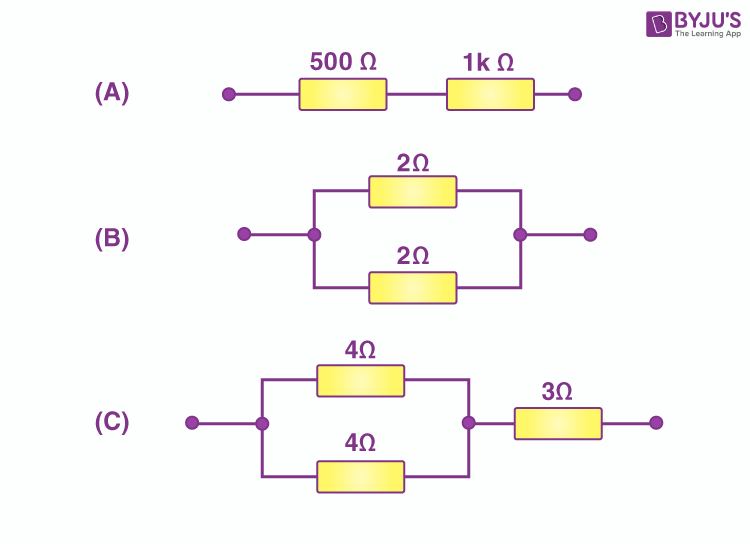a) Given,

R1 = 500Ω

R2 = 1000Ω

From the above circuit diagram,

R = R1 + R2

= 500 + 1000

=1500Ω

b) Given,

R1 = 2Ω

R2 = 2Ω

From the given circuit diagram,

1/R = 1/R1 + 1/R2

1/R = ½ + ½

R = 1Ω

c) Given,

R1 = 4Ω

R2 = 4 Ω

R3 = 3 Ω

From the given circuit diagram,

1/R = 1/R1 + 1/R2

1/R = ¼ + ¼

R = 2 Ω

Total resistance = R + R3

= 2 + 3

= 5 Ω

Q12. Find the current in each resistor in the circuit shown below: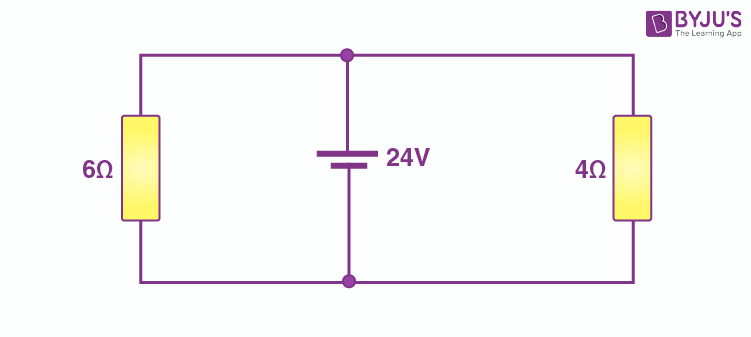Given,

R1 = 6Ω

R2 = 4 Ω

V = 24V

From the given circuit diagram, we know that the two resistances are connected in parallel

Therefore,

Current across R1 = I1 = V/R1 = 24/6 = 4amp

Current across R2 = I2 = V/R2 = 24/4 = 6amp

Q13. Explain with diagrams what is meant by the “series combination” and “parallel combination” of resistance. In this case the resultant resistance is:

a) less

b) more than either of the individual resistance?

Series combination: When two or more resistances are connected end to end, these resistances are said to be connected in a series combination. The sum of all the individual resistances is equal to any number of resistances that are connected in series.

The resultant resistance is usually more than either of the individual resistances.

R = R1 + R2 + ……Parallel combination: When two or more resistances are connected between the same two points, these resistances are said to be connected in parallel combination. The sum of all the individual resistances is equal to the reciprocal of the resistances that are connected in parallel.

The resultant resistance is usually less than either of the individual resistances.

1/R = 1/R1 + 1/R2 + …..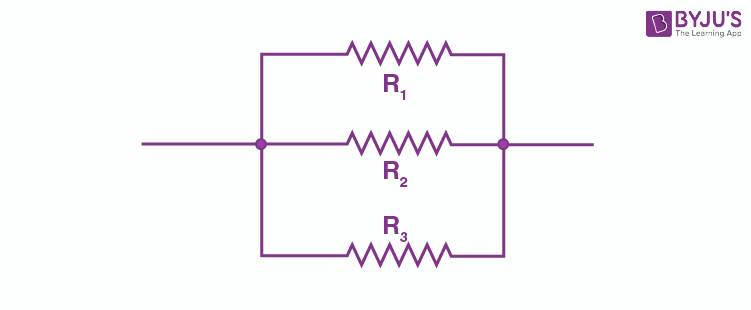Q14. A battery of 9V is connected in series with resistors of 0.2Ω, 0.3 Ω, 0.4 Ω, 0.5 Ω, and 12 Ω. How much current would flow through the 12 Ω resistor?

Given,

R1 = 0.2 Ω

R2 = 0.3 Ω

R3 = 0.4 Ω

R4 = 0.5 Ω

R5 = 12 Ω

V = 9V

Therefore, the resultant resistance is given as:

R = R1 + R2 + R3 + R4 + R5

= 0.2 + 0.3 + 0.4 + 0.5 + 12

= 13.4 Ω

The current flow through 12 Ω resistance is given as: V/R

Therefore, I = 9/13.4

I = 0.67amp

Q15. An electric bulb of resistance 20 Ω and a resistance wire of 4 Ω are connected in series with a 6V battery. Draw the circuit diagram and calculate:

a) total resistance of the circuit

b) current through the circuit

c) potential difference across the electric bulb

d) potential difference across the resistance wire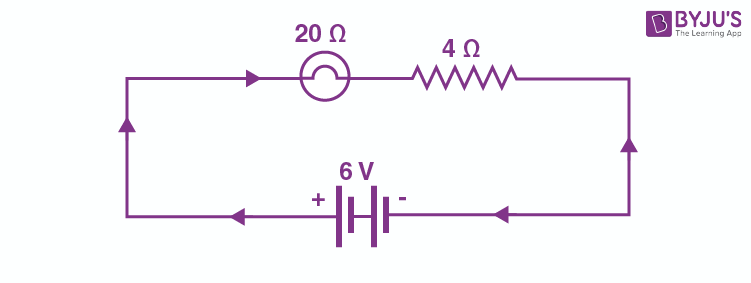a) Total resistance of the circuit is given as:

R = R1 + R2 = 20 + 4 = 24Ω

b) We know that,

V = IR

Therefore,

6 = 24I

I = 6/24 = 0.25amp

c) Potential difference across the bulb = IR1 = 0.25 × 20 = 5V

d) Potential difference across the resistance wire = IR2 = 0.25 × 4 = 1V

Q16. Three resistors are connected as shown in the diagram: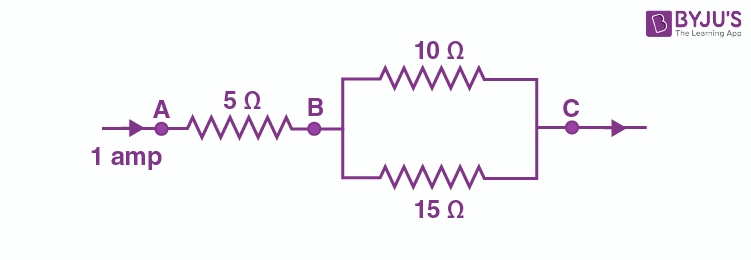Through the resistor 5Ω a current of 1 ampere is flowing.

a) What is the current through the other two resistors?

b) What is the potential difference between AB and across AC?

c) What is the total resistance?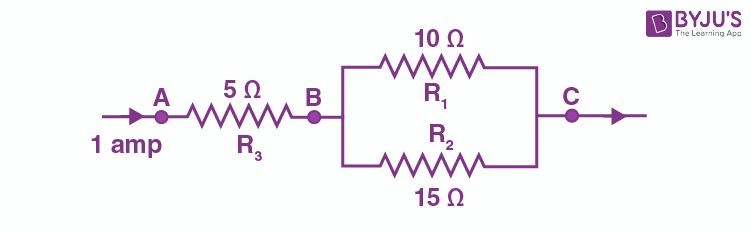From the above circuit diagram;

a) Total current, I entering the resistances R1 and R2 that are arranged in parallel combination is 1amp. Let I1 be the current flow through R1 and I2 be the current flow through R2.

I1 = (IR2)/(R1 + R2)

= (1 × 15)/(10 + 15)

= 0.6A

I2 = (IR1)/(R1 + R2)

= (1 × 10)/ (10 + 15)

= 0.4A

b) Potential difference across AB = IR3 = 1 × 5 = 5V

Equivalent resistance between B and C is

1/R’ = 1/R1 + 1/R2

= 1/10 + 1/15

= 5/30

R’ = 6Ω

The total resistance between A and C is R = 5 + 6 = 11Ω

Potential difference across AC = IR = 1 × 11 = 11V

c) Total resistance = R3 + R’ = 5 + 6 = 11Ω

Q17. For the circuit shown in the diagram below: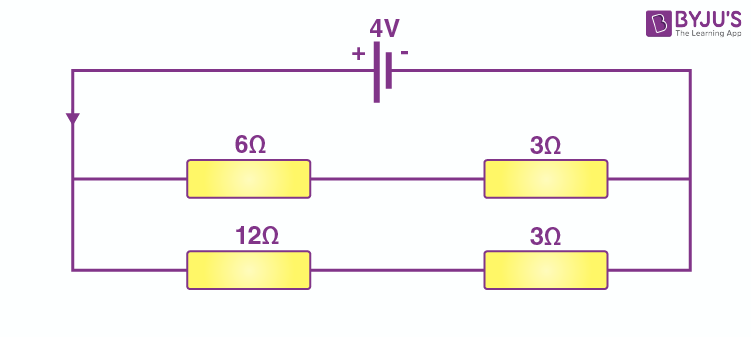What is the value of:

a) Current through 6Ω resistor?

b) Potential difference across 12 Ω resistor?

From the given circuit:

V = 4V

Total resistance in line 1 = R1 = 6 + 3 = 9 Ω

Total resistance in line 2 = R2 = 12 + 3 = 15 Ω

a) Current through 6 Ω resistor is same as the current through line 1 = V/R1 = 4/9 = 0.44 Ω

b) Potential difference across line 2 is 4V

Current through line 2 = V/R2 = 4/15 Ω

Potential difference across 12 Ω resistor = (4/15) × 12 = 3.2V

Q18. Two resistors with resistances 5 Ω and 10 Ω respectively, are to be connected to a battery of emf 6V so as to obtain minimum and maximum current flowing

a) How will you connect the resistances in each case?

b) Calculate the strength of the total current in the circuit in the two cases.

Given,

R1 = 5 Ω

R2 = 10 Ω

V = 6V

a) When the two resistors are connected in series, minimum current flows through the resistors. When the resistors are connected in parallel, the current flow will be maximum.

b) When the resistors are in series,

Total resistance = 5 + 10 = 15Ω

1/R = 1/R1 + 1/R2

1/R = 1/5 + 1/10

1/R = 3/10

R = 10/3 Ω

Therefore, the total current drawn by the circuit = V/R = 6/(10/3) = 1.8amps

Q19. The circuit diagram given below shows the combination of three resistors R1, R2, and R3: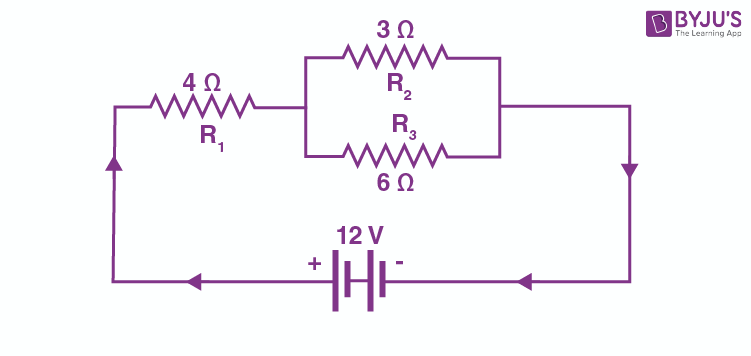Find:

a) total resistance of the circuit

b) total current flowing in the circuit

c) the potential difference across R1

a) Total resistance of two resistors that are connected in parallel is

1/R’ = 1/3 + 1/6

1/R’ = 3/6

R’ = 2 ohms

Total resistance of the circuit = 2 + 4 = 6 ohms

b) Total current flowing through the circuit = V/R

Where R = total resistance

I = 12/6 = 2 amp

c) Potential difference across R1 = R1I = 8V

Q20. In the circuit diagram given below, the current flowing across 5 ohm resistor is 1 amp. Find the current flowing through the other two resistors.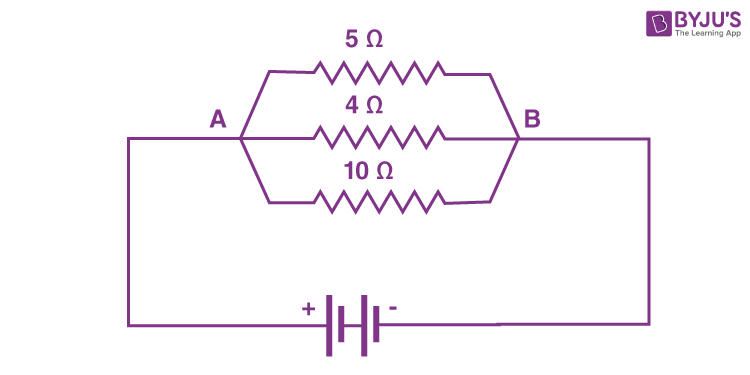Given,

I = 1 amp

R = 5 ohm

The potential difference across each resistor is same as the resistors are connected in parallel and the voltage across these resistors are the same.

Therefore, applied voltage, V = IR = 5V

Current through 4 ohm resistor = V/R = 5/4 = 1.25 amp

Current through 10 ohm resistor = V/R = 5/10 = 0.5 amp

Q21. A resistor has a resistance of 176 ohms. How many of these resistors should be connected in parallel so that their combination draws a current of 5 ampere from a 220 volt supply volt line?

I = 5 amp

V = 220 V

R = V/I = 220/5 = 44 ohm

The resultant resistance must be less than 176 ohm. Therefore, the resistors are connected in parallel

Let the required no be n

Rre = 176/n = 44

N = 176/44 = 4

Q22. An electric heater which is connected to a 220V supply line has two resistance coils A and B of 24 ohm resistance each. These coils can be used separately in series or in parallel. Calculate the current drawn when:

a) only one coil A is used

b) coils A and B are used in series

c) coils A and B are used in parallel

Given,

V = 220V

Ra = Rb = 24 ohm

a) Current drawn when only coil A is used:

I = V/Ra = 220/24 = 9.16 amps

b) Current drawn when the coils A and B are used in series:

Total resistance, R = Ra + Rb = 24 + 24 = 48 ohms

I = V/R = 220/48 = 4.58 amps

c) Current drawn when coils A and B are used in parallel:

Total resistance is 1/R = 1/Ra + 1/Rb = 1/24 + 1/24 = 2/24 = 1/12

R = 12 ohms

I = V/R = 220/12 = 18.33 amps

Q23. In the circuit diagram given below five resistance of 10Ω, 40 Ω, 30 Ω, 20 Ω, and 60 Ω are connected as shown to a 12V battery.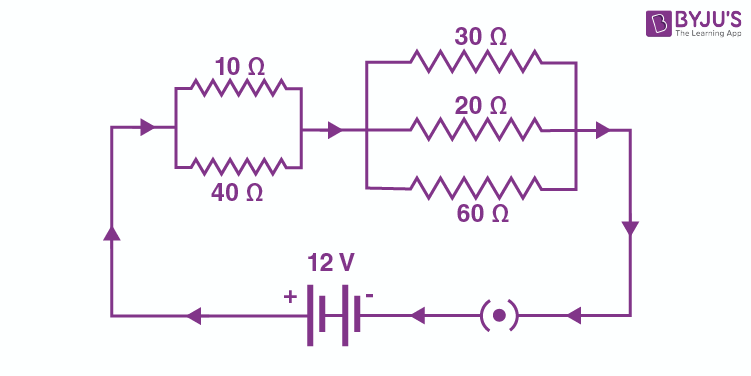Calculate:

a) total resistance in the circuit

b) total current flowing in the circuit

a) Equivalent resistance of 10Ω and 40 Ω resistances is R1 is given as:

1/R1 = 1/10 + 1/40 = 5/40

R1 = 8 Ω

The equivalent resistance of 30 Ω, 20 Ω, and 60 Ω resistance is R2 is given as:

1/R2 = 1/30 + 1/20 + 1/60 = 6/60

R2 = 10 Ω

R1 and R2 are connected in series

Therefore, total resistance in the circuit, R = R1 + R2 = 8 + 10 = 18 Ω

b) Total current flowing in the circuit, I = V/R = 12/18 = 0.67amp

Q24. In the circuit diagram given below, three resistors R1, R2, and R3 of 5 , 10 Ω, and 30 Ω respectively, are connected as shown: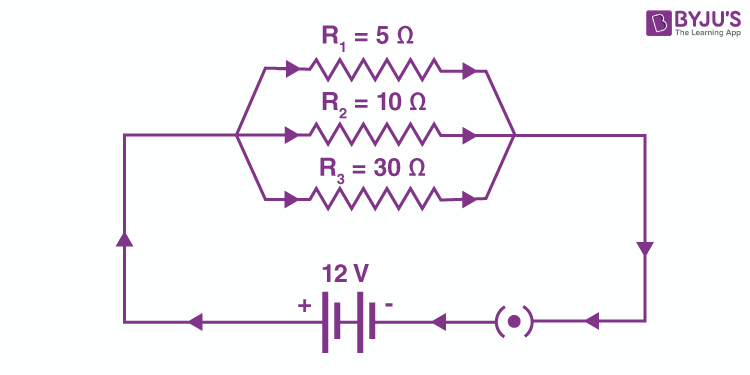Calculate:

a) current through each resistor

b) total current in the circuit

c) total resistance in the circuit

Given,

V = 12V

R1, R2, and R3 are connected in parallel

a) Current through R1 = V/R1 = 12/5 = 2.4 A

Current through R2 = V/R2 = 12/10 = 1.2 A

Current through R3 = V/R3 = 12/30 = 0.4A

b) Total current in the circuit = 2.4 + 1.2 + 0.4 = 4A

c) Total resistance in the circuit = R

1/R = 1/R1 + 1/R2 + 1/R3

1/R = 1/5 + 1/10 + 1/30

1/R = 10/30

R = 3 ohm

Q25. A potential difference of 4V is applied to two resistors of 6Ω and 2 Ω connected in series. Calculate:

a) the combined resistance

b) the current flowing

c) the potential difference across the 6 Ω resistor

Given,

V = 4V

R1 = 6 Ω

R2 = 2 Ω

a) Combined resistance, R = R1 + R2 = 8 ohm

b) Current flowing, I = V/R = 4/8 = 0.5 amp

c) Potential difference across 6 ohm resistor = IR1 = 3V

Q26. A potential difference 6V is applied to two resistors of 3 Ω and 6 Ω connected in parallel. Calculate:

a) the combined resistance

b) the current flowing in the main circuit

c) the current flowing in the 3 Ω resistor

Given,

V = 6V

R1 = 3 Ω

R2 = 6 Ω

a) Combined resistance,

1/R = 1/R1 + 1/R2 = 1/3 + 1/6 = ½

R = 2 Ω

b) Current flowing in the main circuit, I = V/R = 6/2 = 3A

c) Current flowing in 3 Ω resistor = V/R1 = 6/3 = 2A

Q27. In the circuit shown below, the voltmeter reads 10V.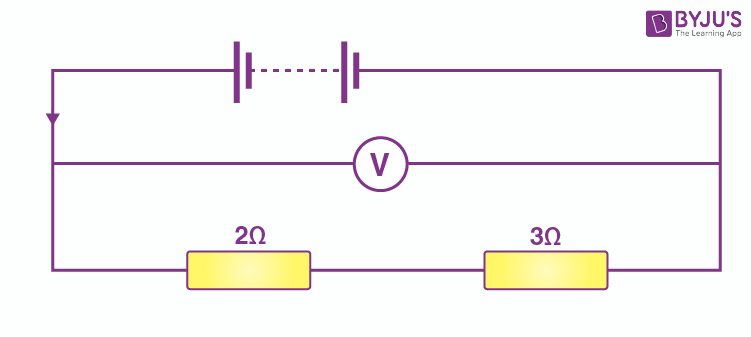a) What is the combined resistance?

b) What current flows?

c) What is the potential difference across 2Ω resistor?

d) What is the potential difference across 3 Ω resistor?

Given,

V = 6V

R1 = 2 Ω

R2 = 3 Ω

a) Combined resistance, Rt = 2+3 = 5 Ω

b) I = V/Rt = 10/5 = 2A

c) Potential difference across 2 Ω resistor = IR1 = 4V

d) Potential difference across 3 Ω resistor = IR2 = 6V

Q28. In the circuit given below: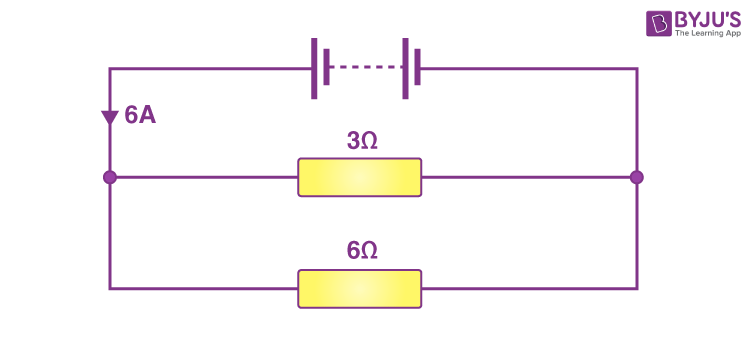a) What is the combined resistance?

b) What is the potential difference across the combined resistance?

c) What is the potential difference across the 3Ω resistor?

d) What is the current in the 3 Ω resistor?

e) What is the current in the 6 Ω resistor?

Total current flowing through circuit, I = 6A

R1 = 3 Ω

R2 = 6 Ω

a) Combined resistance R is

1/R = 1/3 + 1/6 = 3/6

R = 2 Ω

b) Potential difference across the combined resistance = IR = 12V

c) Potential difference across the 3 Ω resistor = Potential difference across the combined resistance = 12V

d) Current flowing through the 3 Ω resistor = V/R1 = 12/3 =4A

e) Current flowing through the 6 Ω resistor = V/R2 = 12/6 = 2A

Q29. A 5V battery is connected to two 20 resistors which are joined together in series.

a)  Draw a circuit diagram to represent this. Add an arrow to indicate the direction of conventional current flow in the circuit.

b) What is the effective resistance of the two resistors?

c) Calculate the current that flows from the battery

d) What is the potential difference across each resistor?

a)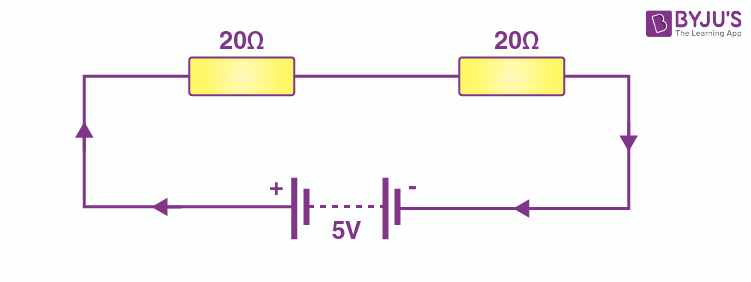b) Effective resistance = 20 + 20 = 40 ohms

c) Current flowing through the circuit = I = V/R = 5/40 = 0.125amp

d) Potential difference across each resistance = IR = 2.5V

Q30. The figure given below shows an electric circuit in which current flows from a 6V battery through two resistors.

a) Are the resistors connected in series with each other or in parallel?

b) For each resistor, state the potential difference across it.

c) The current flowing from the battery is shared between the two resistors. Which resistor will have bigger share of the current?

d) Calculate the effective resistance of the two resistors.

e) Calculate the current that flows from the battery.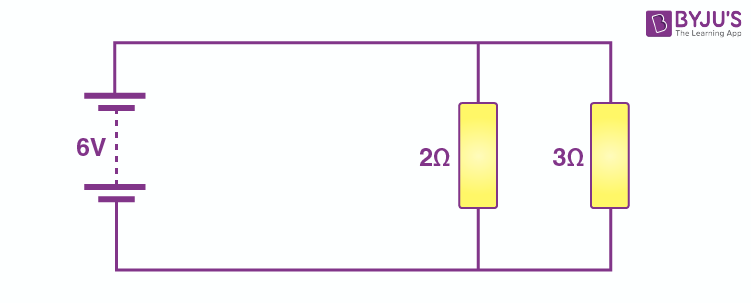Given,

V = 6V

R1 = 2Ω

R2 = 3 Ω

a) Resistors are connected in parallel

b) Potential difference across each resistor is the same and is equal to 6V

c) 2 Ω resistance have bigger share of current because of its lower resistance

d) Effective resistance = R

1/R = ½ + 1/3

1/R = 5/6

R = 1.2 Ω

e) Current flowing through battery, I = V/R = 6/1.2 = 5A

Q31. A 4 Ω coil and a 2 Ω coil are connected in parallel. What is their combined resistance? A total current of 3A passes through the coils. What current passes through the 2 coil?

4 Ω and 2 Ω coils are connected in parallel.

Therefore, the combined resistance, R

1/R = ¼ + ½ = ¾

R = ¾ Ω

Total current,, I = V/R = 3 Ω

V/(4/3) = 3

V = 4V

Current through 2 Ω coil = V/2 = 4/2 = 2 A

Q32. a) With the help of a circuit diagram, deduce the equivalent resistance of two resistances connected in series.

b) Two resistances are connected in series, as shown in the diagram: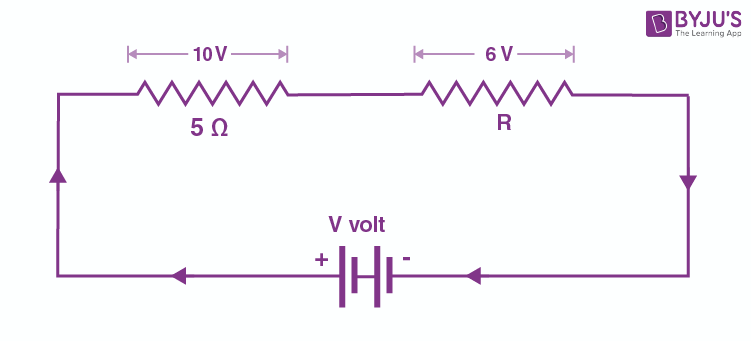i) What is the current through the 5 ohm resistance?

ii) What is the current through R?

iii) What is the value of R?

iv) What is the value of V?

a)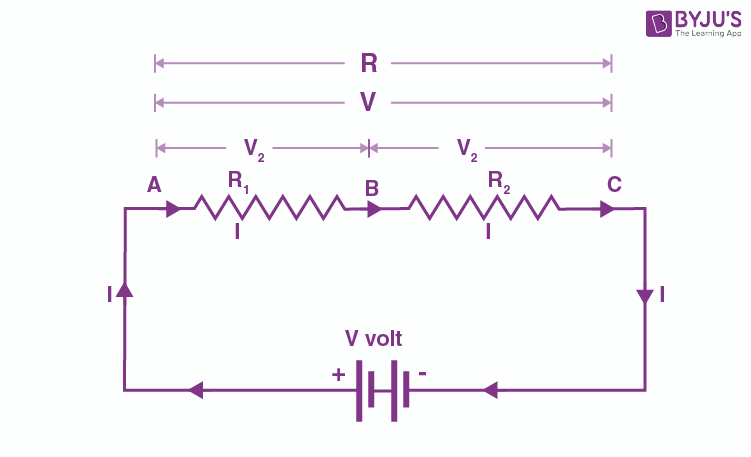Above circuit shows resistances R1 and R2 connected in series with a battery of V volts

Let the potential difference across R1 be V1 and the potential difference across R2 be V2

V = V1 + V2 (1)

By Ohm’s law

R = V/I

V = IR (2)

Applying Ohm’s law

V1 = IR1 (3)

V2 = IR2 (4)

From (1), (2), (3), and (4)

R = R1 + R2

b) i) Current through 5 ohm resistor = 2A

ii) Current through R = 2A because 5 ohm resistor and R are connected in series

iii) V = IR

R = 3 ohm

iv) V = 10 + 6 = 16V

Q33. a) With the help of a diagram, derive the formula for the resultant resistance of three resistors connected in series.

b) For the circuit shown in the diagram given below, calculate

i) Value of current through each resistor

ii) total current in the circuit

iii) total effective resistance of the circuit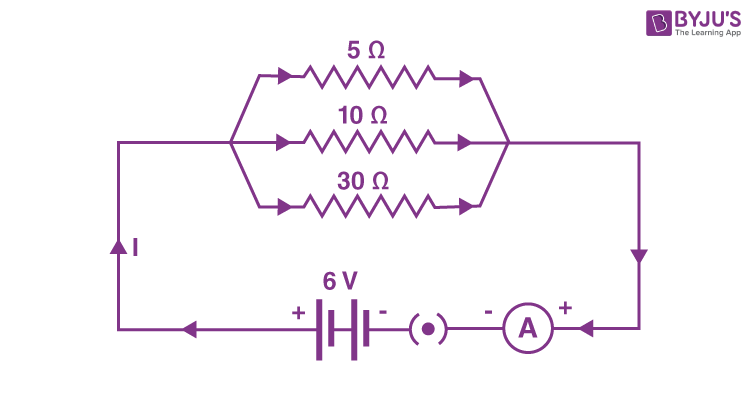a)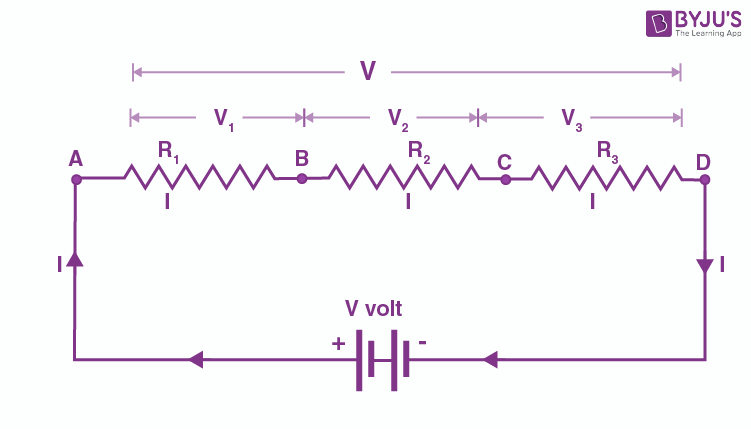We know that V = V1 + V2 + V3

R = V/I

V1 = IR1

V2 = IR2

V3 = IR3

Using the above equations,

IR = IR1 + IR2 + IR3

R = R1 + R2 + R2

b) i) Current through R1 = 1.2A

Current through R2 = 0.6A

Current through R3 = 0.2A

ii) Total current in the circuit = 2A

iii) Effective resistance = 3 ohm

Q34. a) With the help of a circuit diagram, obtain the relation for the equivalent resistance of two resistance connected in parallel. Find

i) Total resistance

ii) Current shown by the ammeter A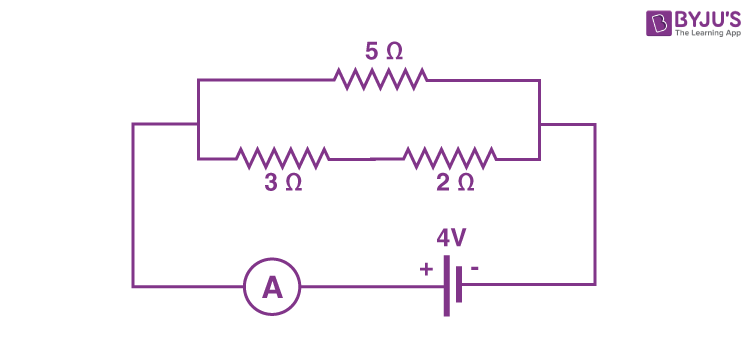a)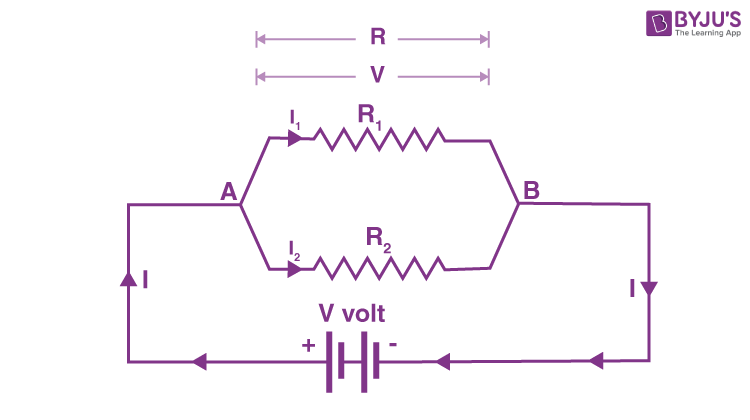Total current = I = I1 + I2

I1 = V/R1

I2 = V/R2

V/R = V/R1 + V/R2

1/R = 1/R1 + 1/R2

b) i) Total resistance = R

R1 = 5 ohms

R2 = 3+2 = 5 ohms

R = 2.5 ohms

ii) Current flowing through the circuit, I = V/R = 1.6 amps

Q35. a) Explain with the help of a labelled circuit diagram, how you will find the resistance of a combination of three resistors of resistance R1, R2, and R3 joined in parallel

b) In the diagram shown below, the cell and the ammeter both have negligible resistance. The resistors are identical.

With the switch K open, the ammeter reads 0.6A. What will be the ammeter reading when the switch is closed?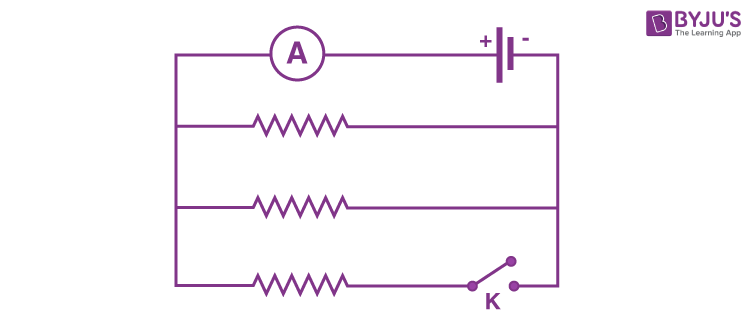a)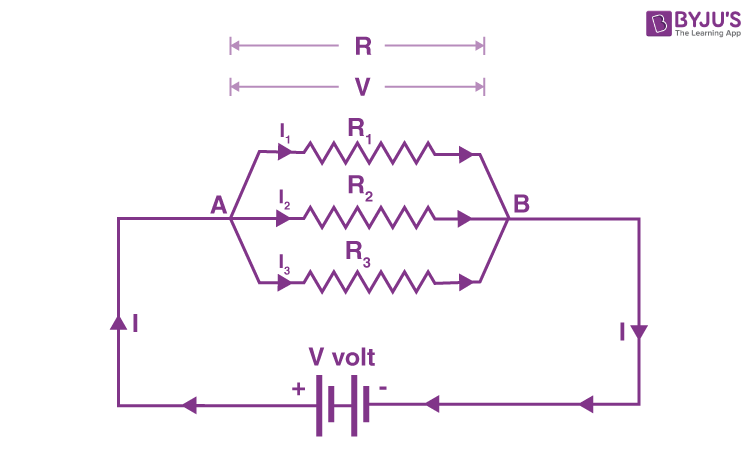I = V/R

I1 = V/R1

I2 = V/R2

I3 = V/R3

1/R = 1/R1 + 1/R2 + 1/R3

b) Effective resistance = 1/R + 1/R = 2/R

Current, I = V/(R/2) = 0.6A

V/R = 0.3A

Therefore, final current, I = V/(R/3) = 0.9A

Multiple Choice Questions

Q36. The figure given below shows three resistors: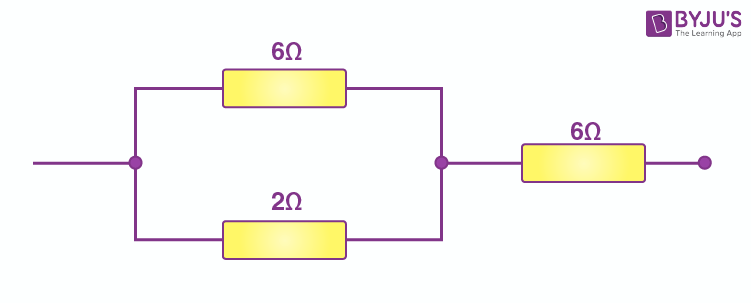Their combined resistance is:

a) 1 (5/7)Ω

b) 14 Ω

c) 6 (2/3) Ω

d) 7 (1/2) Ω

The correct option is d) 7 (1/2) Ω

When the resistors are in combination, series and parallel combination formula needs to be used. Resistors with 6 Ω and 2 Ω are in parallel combination. Their resultant resistance is then added with 6 Ω resistor to obtain the total resistance of the circuit.

Q37. If two resistors of 25 Ω and 15 Ω are joined together in series and then placed in parallel with a 40 Ω resistor, the effective resistance of the combination is:

a) 0.1 Ω

b) 10 Ω

c) 20 Ω

d) 40 Ω

The correct option is c) 20 Ω

The resultant resistance obtained from the resistors that are placed in series combination is connected with 40 Ω in parallel such that the overall resistance obtained is equal to 20 Ω.

Q38. The diagram below shows part of a circuit: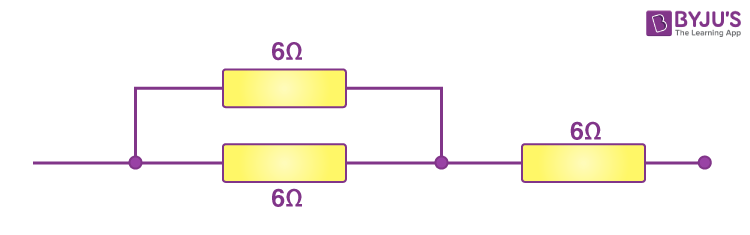If this arrangement of three resistors was to be replaced by a single resistor, its resistance should be:

a) 9Ω

b) 4 Ω

c) 6 Ω

d) 18 Ω

The correct option is a) 9 Ω

Again series and parallel combination are used for the calculation of the overall resistance of the given circuit.

Q39. In the circuit shown below: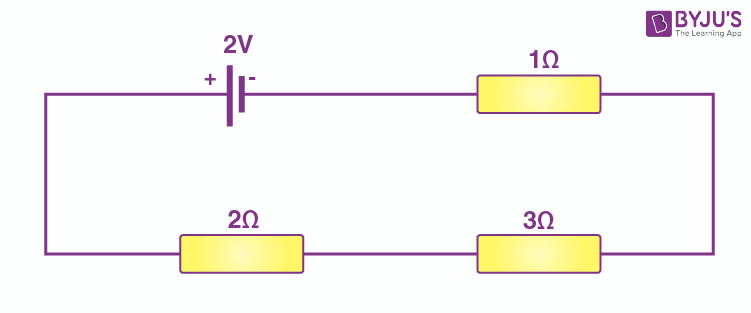The potential difference across the 3Ω resistor is:

a) 1/9 V

b) 1/2V

c) 1V

d) 2V

The correct option is c) 1V

We know that the potential difference across a resistor is calculated as V = IR. Therefore, by substituting the values, we get potential difference across the resistor of 3Ω as 1V.

Q40. A battery and three lamps are connected as shown: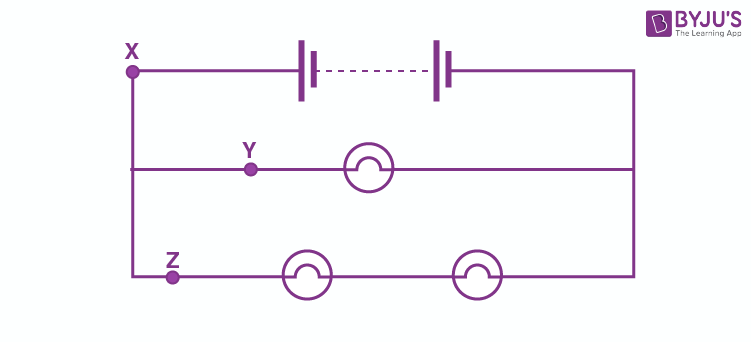Which of the following statements about the currents at X, Y, and Z is correct?

a) The current at Z is greater than that at Y

b) The current at Y is greater than that at Z

c) The current at X equals the current at Y

d) The current at X equal the current at Z

The correct option is b) The current at Y is greater than that at Z

Q41. V1, V2, and V3 are the potential difference across the 1 Ω, 2 Ω, and 3 Ω resistors in the following diagram, the current is 5A.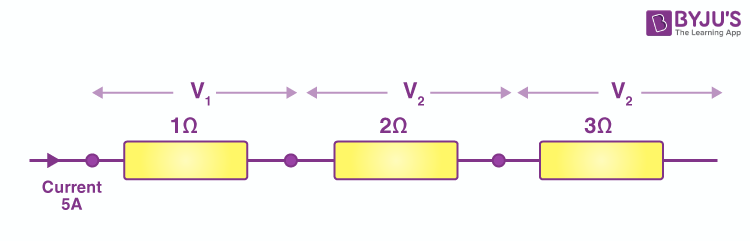Which one of the columns (a) to (d) shows the correct values of V1, V2, and V3 measured in volts?

 V1 V2 V3 a) 1.0 2.0 3.0 b) 5.0 10.0 15.0 c) 5.0 2.5 1.6 d) 4.0 3.0 2.0

The correct option is b) Where V1 = 5.0, V2 = 10.0, and V3 = 15.0

Using the formula, V = IR, substituting the values in the formula, we get the correct values for V1, V2, and V3.

Q42. A wire of resistance R1 is cut into five equal pieces. These five pieces of wire are then connected in parallel. If the resultant resistance of this combination be R2, then the ratio R1/R2 is:

a) 1/25

b) 1/5

c) 5

d) 25

The correct option is d) 25.

When a resistance wire is cut into five pieces, the ratio of the resistance and the resultant resistance obtained when the pieces are connected in parallel is 25.

Page No: 47

Q1. Are the lights in your house wires in series?

The light in our houses are not connected in series. They are connected in parallel.

Q2. What happens to the other bulbs in a series circuit if one bulb blows off?

When the bulbs are connected in series, all the other bulbs stop glowing.

Q3. What happens to the other bulbs in a parallel circuit if one bulb blows off?

When the bulbs are in parallel, they continue to glow.

Q4. Which type of circuit, series or parallel, is preferred while connecting a large number of bulbs:

a) for decorating a hotel building from outside?

b) for lighting inside the rooms of the hotel?

The following types of circuits are used when:

a) hotel building is to be decorated from outside: series

b) lighting the rooms inside the hotel: parallel

Q5. Draw a circuit diagram to show how two 4V electric lamps can be lit brightly from two 2V cells.

Following is the circuit diagram of two lamps with 4V each and arranged in parallel with 2V cells:Q6. Why is a series arrangement not used for connecting domestic electrical appliances in a circuit?

A series arrangement is not used for connecting domestic electrical appliances in a circuit because if any of the electrical appliances stops working, it affects all the other appliances.

Q7. Give three reasons why different electrical appliances in a domestic circuit are connected in parallel.

Different electrical appliances in a domestic circuit are connected in parallel because:

a) the voltage distribution to each appliance is the same from the power supply line

b) the working of the appliance can be controlled using individual switch

c) the working of the appliances are not dependent on each other

Q8. Ten bulbs are connected in a series circuit to a power supply line. Ten identical bulbs are connected in a:

a) an identical power supply line

b) which circuit would have the highest voltage across each bulb?

c) in which circuit would the bulbs be brighter?

d) in which circuit, if one bulb blows out, will all others stop glowing?

e) which circuit would have less current in it?

a) To have an identical power supply line, the circuit must be parallel

b) When the circuit is parallel, each bulb will have the highest voltage

c) The working of the appliance can be controlled using an individual switch when a parallel circuit is used

d) The working of the appliances are not dependent on each other when they are connected in parallel

Q9. Consider the circuits given below: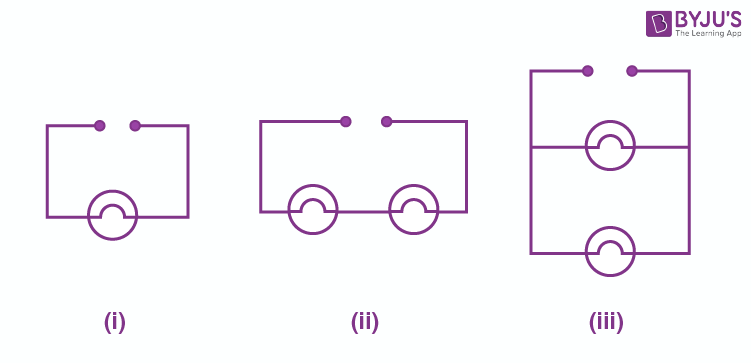a) In which circuit are the lamps dimmest?

b) In which circuit or circuits are the lamps of equal brightness to the lamps in the circuit?

c) Which circuit gives out the maximum light?

a) In circuit (ii) the lamps are the dimmest

b) In circuit (iii) the lamps are of equal brightness

c) In circuit (i) the maximum light is obtained

Q10. If you were going to connect two light bulbs to one battery, would you use a series or a parallel arrangement? Why? Which arrangement takes more current from the battery?

When the arrangement is in parallel the consumption of current from the battery is more. The defect in any of the bulb won’t affect the working of the other bulbs.

Q11. a) Which is the better way to connect lights and other electrical appliances in domestic wiring: series circuits or parallel circuits? Why?

b) Christmas tree lamps are usually wired in series. What happens if one lamp breaks?

c) An electrician has wired a house in such a way that if a lamp gets fused in one room of the house, all the lamps in other rooms of the house stop working. What is the defect in the wiring?

d) Draw a circuit diagram showing two electric lamps connected in parallel together with a cell and a switch that works both lamps. Mark an A enclosed in a circle on your diagram to show where an ammeter should be placed to measure the current.

a) When domestic wiring is to be done, parallel circuits are better than series. This is because when one of the electrical appliances stops working, others are affected.

b) When the lamps are connected in series, the lamps stop glowing.

c) All the lamps are connected in series.

d)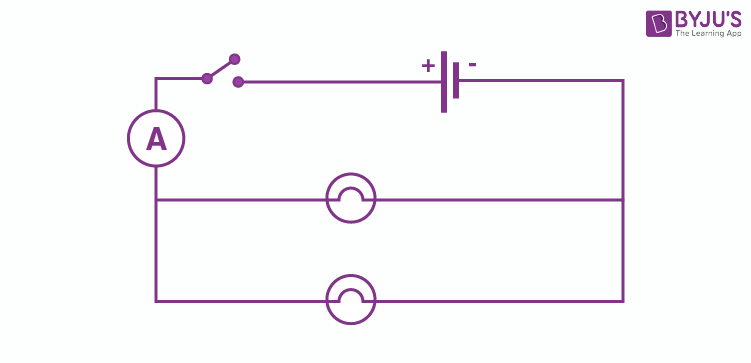Multiple Choice Questions

Q12. The lamps in a household circuit are connected in parallel because:

a) this way they required less current

b) if one lamp fails, the others remain lit

c) this way they require less power

d) if one lamp fails the others also fail

The correct option is b) if one lamp fails, the others remain lit.

When the lamps are arranged in parallel connection, the current flowing through them are different. Therefore, if one lamp fails, the others remain lit.

Q13. Using the circuit given below, state which of the following statement is correct.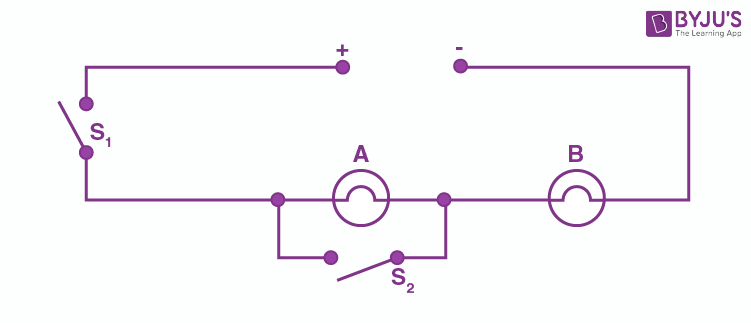a) When S1 and S2 are closed, lamps A and B are lit

b) With S1 open and S2 closed, A is lit and B is not lit

c) With S2 open and S1 closed A and B are lit

d) With S1 closed and S2 open, lamp A remains lit even if lamp B gets fused

The correct option is c) With S2 open and S1 closed A and B are lit.

High Order Thinking Skills

Q14. a) Draw a circuit diagram showing two lamps, one cell, and a switch connected in series.

b) How can you change the brightness of the lamps?

a)b) The brightness of the lamp can be changed by connecting the lamps in parallel.

Q15. Consider the circuit given below, where A, B, and C are three identical light bulbs of constant resistance.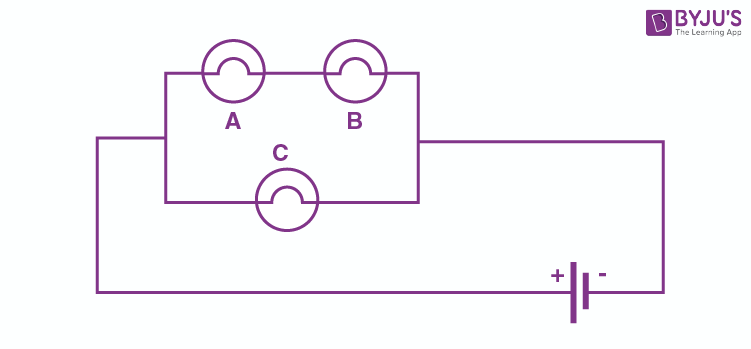a) List the bulbs in order of increasing brightness

b) If C burns out, what will be the brightness of A now compared with before?

c) If B burns out instead, what will be the brightness of A and C compared with before?

a) The brightest among the three is C, while A and B will have equal brightness.

b) The brightness of A will remain unchanged if C burns out as the voltage of A remains unaltered.

c) If B burns out, then A will also burn out as they are connected in series with each other. However, C remains to be the brightest.

Q16. How do you think the brightness of two lamps arranged in parallel compares with the brightness of two lamps arranged in series?

When the two lamps are arranged in parallel, the lamps will have more brightness than when they are arranged in series.

Q17. If current flows through two lamps arranged in:

a) series

b) parallel

and the filament of one lamp breaks, what happens to the other lamp? Explain

a) When the lamps are arranged in series, the lamps stop glowing when the filament of one of the lamps breaks.

b) When the lamps are arranged in parallel, the lamps continue to glow even if one of the lamps breaks.

Q18. The figure below shows a variable resistor in a dimmer switch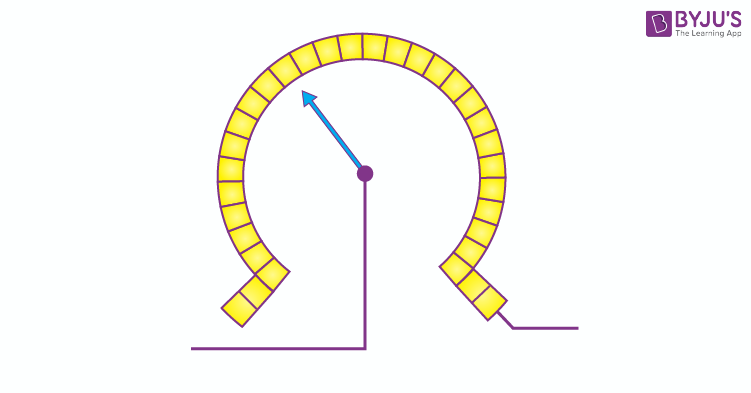How would you turn the switch to make the lights

a) brighter

b) dimmer

a) To make the lights brighter, the switch has to be turned to the right as the resistance decreases when turned to the right.

b) To make the lights dimmer, the switch has to be turned to the left as the resistance increases when turned to the left.

Page No: 58

Q1. State two factors on which the electrical energy consumed by an electrical appliance depends.

The following are the two factors on which the electrical energy consumed by an electrical appliance depends:

a) Usage time of the appliance

b) Power rating of the appliance

Q2. Which one has a higher electrical resistance: a 100 watt bulb or a 60 watt bulb?

60 watt bulb has a higher electrical resistance than a 100 watt bulb because we know that power is inversely proportional to the resistance.

Q3. Name the commercial unit of electric energy.

The commercial unit of electric energy is kilowatt-hour.

Q4. An electric bulb is rated at 220V, 100W. What is its resistance?

Given,

V = 220V

P = 100W

R = ?

We know that,

R = V2/P

R = 484 ohm

Q5. What is the SI unit of electric energy and electric power?

The SI unit of electric energy is joule and the SI unit of electric power is watt.

Q6. Name the quantity whose unit is kilowatt and kilowatt-hour.

Kilowatt is the unit of electric power and kilowatt-hour is the unit of electric energy.

Q7. Which quantity has the unit of watt?

Watt is the unit of electric power.

Q8. What is the meaning of the symbol kWh? Which quantity does it represent?

kWh is the commercial unit of electrical energy and it stands for kilowatt-hour.

Q9. If the potential difference between the end of a wire of fixed resistance is doubled, by how much does the electric power increase?

We know that,

P =V2/R

R is constant

V is doubled

Therefore, P = 2(V2)/R

P = 4V/R

Therefore, when the resistance is doubled, power becomes four times the actual value.

Q10. An electric lamp is labelled 12V, 36W. This indicates that it should be used with a 12V supply. What other information does the label provide?

The other information provided by the label is that the rate of consumption of energy takes place at 36J/s.

Q11. What current will be taken by a 920W appliance if the supply voltage is 230V?

Given,

P = 920W

V = 230V

I = ?

We know that,

P = VI

920 = 230I

I = 920/230

I = 4A

Q12. Define watt. Write down an equation linking watts, volts, and ampere.

Following is the equation that relates watts, volts, and ampere:

1 watt = 1 volt × 1 ampere

Q13. Define watt-hour. How many joules are equal to 1 watt-hour?

Watt-hour is defined as the amount of electrical energy that is consumed when an electrical appliance of 1 watt power is used for 1 hour.

1 watt-hour = 3600 joules.

Q14. How much energy is consumed when a current of 5 ampere flows through the filament of a heater having resistance of 100 ohms for two hours? Express it in joules.

Given,

I = 5A

R = 100 ohm

T = 2 hours

We know that,

Energy consumed = P × T = I2RT

Substituting the values,

Energy consumed = 5kWh

1kWh = 3.6 × 106J

Therefore, 5kWh = 5 ×3.6 × 106J = 18 × 106J

Q15. An electric bulb is connected to a 220V power supply line, if the bulb draws a current of 0.5A, calculate the power of the bulb.

Given,

V = 220V

I = 0.5A

P = ?

We know that,

P = VI

P = 110 watt

Q16. In which of the following cases more electrical energy is consumed per hour?

a) A current of 1 ampere passed through a resistance of 300 ohms

b) A current of 2 amperes passed through a resistance of 100 ohms

a) Given,

R = 300 ohm

I = 1 amp

T = 1 hour

P = I2R = 300W

E = PT = 300 watt-hour

b) Given,

R = 100 ohm

I = 2 amp

T = 1 hour

P = I2R = 400W

E = PT = 400 watt-hour

Therefore, in the second case, the consumption of electrical energy per hour is more.

Q17. An electric kettle rated at 220V, 2.2kW works for 3 hours. Find the energy consumed and the current drawn.

Given,

V = 220V

P = 2.2kW = 2200 watt

T = 3 hours

We know that

Energy consumed = PT = 6.6kWh

We also know that,

P = VI

Therefore, I = 10 amp

Q18. In a house, two 60W electric bulbs are lighted for 4 hours and three 100W bulbs for 5 hour every day. Calculate the electric energy consumed in 30 days.

Case 1

Power, P1 = 60W

Number, n1 = 2

Time for use, T1 = 4 hours everyday

Energy consumed, E1 = n1 × P1 × T1

E1 = 2 × 60 × 4 = 480watt-hour = 0.48kWh

Therefore, energy consumed for 30 days = 30 × 0.48 = 14.4watt-hour

Case 2

Power, P2 = 100W

Number, n2 = 3

Time for use T2 = 5 hours everyday

Energy consumed, E2 = n2 × P2 × T2

E2 = 3 × 100 × 5 = 1500 = 1.5kWh

Therefore, energy consumed for 30 days = 30 × 1.5 = 45kWh

Therefore, overall energy consumed = 14.4 + 45 = 59.4kWh

Q19. A bulb is rated as 250V, 0.4A. Find

a) Power

b) Resistance

Given,

V = 250V

I = 0.4 amp

a) P = ?

P = VI

P = 100 watt

b) R = ?

P = I2R

R = 625 ohm

Q20. For a heater rated at 4kW and 220V, calculate:

a) the current

b) the resistance of the heater

c) the energy consumed in 2 hours

d) the cost if 1 kWh is priced at Rupees 60

Given,

P = 4kW

V = 220V

a) I = ?

P = VI

I = 16amp

b) R = ?

P = I2R

R = 15.25 ohm

c) Energy consumed in two hours = PT

E = 8kWh

d) If 1kWh = Rupees 4.6

Total cost = Rupees 36.8

Q21. An electric motor takes 5 ampere current from a 220 volt supply line. Calculate the power of the motor and electric energy consumed by it in 2.

Given,

I = 5amp

V = 220V

T = 2 hours

P = ?

E = ?

P = VI

P = 1.1kW

Energy consumed E = PT

E = 2.2kWh

Q22. Which uses more energy: a 250W TV set in 1 hour or a 1200W toaster in 10 minutes?

Case 1: TV set

P = 250W = 0.25kWh

T = 1 hour

Energy consumed, E = PT = 0.25kWh

Case 2: Toaster

P = 1200W = 1.2kW

T = 10 min = 1/6 hour

Energy consumed, E = PT = 0.2kWh

Therefore, it can be concluded that TV uses more power.

Q23. Calculate the power used in the 2Ω resistor in each of the following circuits:

a) a 6V battery in series with 1Ω and 2Ω resistors

b) a 4V battery in parallel with 12Ω and 2Ω resistors

a) Given,

V = 6V

R1 = 1 ohm

R2 = 2 ohm

Equivalent resistance, R = R1 + R2 = 3 ohm

Total current, I = V/R = 6/3 = 2amp

Current through R2 = I2 = I = 2amp

Voltage across R2 = V2 = I2R2 = 4 ohm

Power used in R2 = I2V2 = 8W

b) V = 4V

R1 = 12 ohm

R2 = 2 ohm

Voltage across R2 = V2 = V = 4V

Current across R2 = I2 = V2/R2 = 2 amp

Power used in R2 = I2V2 = 8W

Q24. Two lamps one rated 40 watt at 220V and the other 60 watt at 220V are connected in parallel to the electric supply at 220V.

a) Draw a circuit diagram to show the connections

b) Calculate the current drawn from the electric supply

c) Calculate the total energy consumed by the two lamps together when they operate for one hour

Given,

No.of lamps = 2

P1 = 40W

P2 = 60W

V = 220V

a)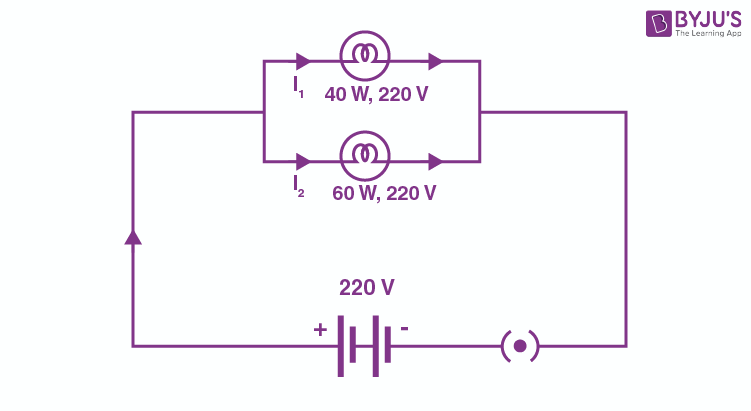b) Voltage across both the bulbs is the same and is equal to 220V

Current through 40W lamp = I1 = P1/V = 40/220 amp

Current through 60W lamp = I2 = P2/V = 60/220 amp

Total current drawn from the electric supply = 40/220 + 60/220 = 0.45 amp

c) Energy consumed by 40W lamp in 1 hour, E1 = PT = 40 watt-hour

1 watt-hour = 3.6kj

E1 = 144kJ

Energy consumed by 60 watt lamp in 1 hour, E2 = PT = 216kJ

Total energy consumed = 144 + 216 = 360kJ

Q25. An electric kettle connected to the 230V mains supply draws a current of 10A. Calculate:

a) the power of the kettle

b) the energy transferred in 1 minute

Given,

V = 230V

I = 10amp

a) P = VI

P = 2300 watt = 2300 J/s

b) Energy consumed in one minute = PT

= 138000 J

Q26. A 2kW heater, a 200 watt TV and three 100 watt lamps are all switched on from 6-10 PM. What is the total cost of Rupees 5.50 per kWh?

For heater,

P = 2kWh

T = 4 hours

Energy consumed = PT = 8kWh

For TV,

P = 200 watt = 0.2kW

T = 4 hours

Energy consumed = PT = 0.8kWh

For lamps,

P = 100 watt = 0.1kW

T = 4 hours

n = 3

Energy consumed = PTn = 1.2kWh

Total energy consumed = 8 + 0.8 + 1.2 = 10kWh

Cost of 1kWh = Rupees 5.50

Total cost = Rupees 55.

Q27. What is the maximum power in kilowatts of the appliance that can be connected safely to a 13A, 230V mains socket?

Given,

I = 13amp

V = 230V

P = VI

P = 2.99kW

Q28. An electric fan runs from the 230V mains. The current flowing through it is 0.4A. At what rate is electrical energy transferred by the fan?

Given,

V = 230V

I = 0.4ampRate at which electrical energy is transferred = Power

P = VI

P = 92watt = 92J/s

Q29. a) What is meant by “electric power”? Write the formula for electric power in terms of potential difference and current.

b) The diagram below shows a circuit containing a lamp L, a voltmeter, and an ammeter. The voltmeter reading is 3V, and the ammeter reading is 0.5A.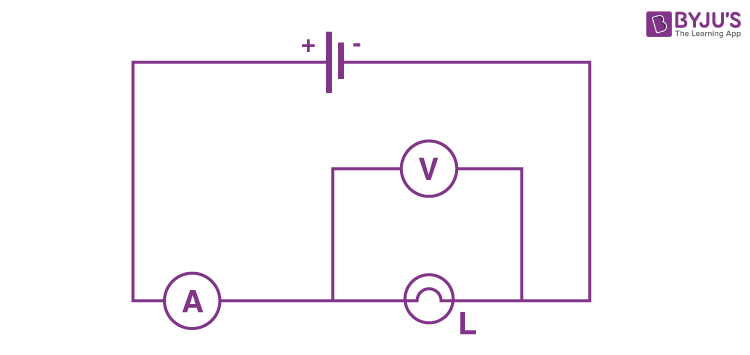What is the resistance and power of the lamp?

c) Define kilowatt-hour. How many joules are there in one kilowatt-hour?

d) Calculate the cost of operating a heater of 500W for 20 hours at the rate of? Rupees 3.90 per unit.

a) Electric power is defined as the rate at which the consumption of electric energy takes place. It is given as:

P = VI = watt

b) Given,

V = 3V

I = 0.5 amp

R = ?

P = ?

V = IR

R = 6 ohms

P = VI

P = 1.5 watt

c) The energy consumed when an electrical appliance with power 1 kilowatt is used for 1 hour, that is known as one kilowatt hour.

d) Given,

P = 500W = 0.5kW

T = 20 hour

Energy consumed = PT = 10kWh

Total cost = ?

Cost per unit = Rupees 3.9

Therefore, total cost = Rupees 39

Multiple Choice Questions

Q30. When an electric lamp is connected to 12V battery, it draws a current of 0.5A. The power of the lamp is:

a) 0.5W

b) 6W

c) 12W

d) 24W

The correct option is b) 6W

We know that power is calculated as P = VI. Substituting the values of V and I we get P = 6W.

Q31. The unit for expressing electric power is:

a) Volt

b) Joule

c) Coulomb

d) Watt

The correct option is d) Watt

Watt is the unit that is used for expressing electric power.

Q32. Which of the following is likely to be the correct wattage for an electric iron used in our homes?

a) 60W

b) 250 W

c) 850W

d) 2000W

The correct option is c) 850W

The electric iron that is used in our homes has 850W power.

Q33. An electric heater is rated at 2kW. Electrical energy costs rupees 4 per kWh. What is the cost of using the heater for 3 hours?

a)  Rupees 12

b) Rupees 24

c) Rupees 36

d) Rupees 48

The correct option is b) Rupees 24.

It is given that the electrical energy cost is Rupees 4/kWh

Time duration is 3 hours

The power of the electric heater is 2kW

Using the given information, we can calculate the amount for 3 hours as Rupees 24.

Q34. The SI unit of energy is:

a) Joule

b) Coulomb

c) Watt

d) Ohm-meter

The correct answer is a) Joule

The SI unit of energy is joule.

Q35. The commercial unit of energy is:

a) Watt

b) Watt-hour

c) Kilowatt-hour

d) Kilo-joule

The correct option is c) Kilowatt-hour

Kilowatt-hour is the commercial unit of energy.

Q36. How much energy does a 100W electric bulb transfer in 1 minute?

a) 100J

b) 600J

c) 3600J

d) 6000J

The correct option is d) 6000J

6000J of energy is transferred in an electric bulb with a power of 100W in 1 minute.

Q37. An electric kettle for use on a 230V supply is rated at 3000W. For safe working, the cable connected to it should be able to carry at least:

a) 2A

b) 5A

c) 10A

d) 15A

The correct option is d) 15A

15A current is required for the safe working of an electric kettle that has a supply of 230V.

Q38. How many joules of electrical energy is transferred per second by a 6V, 0.5A lamp?

a) 30 J/s

b) 12 J/s

c) 0.83 J/s

d) 3 J/s

The correct option is d) 3 J/s

When 6V and 0.5A lamp is used, 3 J/s electrical energy is required.

Q39. At a given time, a house is supplied with 100A at 220V. How many 75W, and 220V light bulbs could be switched on in the house at the same time if they are all connected in parallel?

a) 93

b) 193

c) 293

d) 393

The correct option is c) 293

A total of 293 bulbs can be used when they are all connected in parallel and have 75W and 220V.

Q40. If the potential difference between the ends of a fixed resistor is halved, the electric power will becomes:

a) double

b) half

c) four times

d) one-fourth

The correct option is d) one-fourth

When the potential difference between the ends of a fixed resistor is halved, the electric power becomes one-fourth of the actual value.

Lakhmir Singh Physics Class 10 Solutions Page No: 66

Q1. How does the heat H produced by a current passing through a fixed resistance wire depend on the magnitude of current I?

The heat H produced by a current passing through a fixed resistance wire is directly proportional to the magnitude of the current I.

Q2. If the current passing through a conductor is doubled, what will be the change in heat produced?

When the current passing through a conductor is doubled, the heat produced will increase by four times. This is because the heat produced is directly proportional to the square of the current.

Q3. Name two effects produced by electric current.

Following are the two effects produced by electric current:

a) Heating effect

b) Magnetic effect

Q4. Which effect of current is utilized in an electric light bulb?

The heating effect of current is utilized in an electric light bulb.

Q5. Which effect of current is utilized in the working of an electric fuse?

The heating effect of current is utilized in the working of an electric fuse.

Q6. Name two devices which work on the heating effect of electric current.

Following are the two devices that work on the heating effect of an electric current:

a) Electric heater

b) Electric fuse

Q7. Name two gases which are filled in filament-type electric light bulbs.

Following are the two gases that are filled in filament-type electric light bulbs:

a) Argon

b) Nitrogen

Q8. Explain why filament-type electric bulbs are not power efficient.

Filament-type electric bulbs are not power efficient because they consume most of the electric power as heat and a very small amount of electric power is converted into light.

Q9. Why does the connecting cord of an electric heater not glow hot while the heating element does?

The connecting cord of an electric heater does not glow hot, while the heating element does because the connecting cord is made from copper, which does not glow as the heat produced is negligible. Whereas a heating element made from the nichrome glows because a large amount of heat is produced when the current is passed through it.

Q10. a) Write down the formula for the heat produced when a current I is passed through a resistor R for time t.

b) An electric iron of resistance 20 ohms draws a current of 5 amperes. Calculate the heat produced in 30 seconds.

a) Following is the formula for the heat produced:

H = I2Rt

b) Given,

R = 20Ω

I = 5amp

t = 30s

We know that,

H = I2Rt

Substituting the values we get,

H = 15000J

Q11. State three factors on which the heat produced by an electric current depends. How does it depend on these factors?

Following are the three factors on which the heat produced by an electric current depends:

a) Heat produced is directly proportional to the square of current

b) Heat produced is directly proportional to resistance

c) Heat produced is directly proportional to the time for which the current flows

Q13. Why is an electric bulb not filled with air? Explain why argon or nitrogen is filled in an electric bulb.

An electric bulb is not filled with air because the filament in an electric bulb is made using tungsten which burns quickly when it comes in contact with the oxygen in the air. This is the reason why unreactive gases such as argon and nitrogen are used for filling an electric bulb. These gases are chemically unreactive.

Q14. Explain why tungsten is used for making the filaments of an electric bulb.

Tungsten is used for the making of the filaments of an electric bulb because the melting point of the tungsten is very high. This means that the filament remains burning hot but won’t melt. The other reason for using tungsten is that it is flexible, and the rate of evaporation is low at high temperatures.

Q15. Explain why the current that makes the heater element very hot, only slightly warms the connecting wires leading to the heater.

The connecting wires leading to the heater are slightly warmer than the wires, which makes the heater element hot because they have low resistance, which results in the flow of negligible heat production in them.

Q16. When a current of 4amp passes through a certain resistor for 10 minutes, 2.88 × 104 J of heat are produced. Calculate

a) the power of the resistor

b) the voltage across the resistor

Given,

I = 4amp

T = 10min = 600s

H = 2.88 × 104 J

a) We know that,

H = I2RT

28800 = 42×600R

R = 3 ohms

We know that,

P = I2R

P = 48W

b) We know that,

V = IR

V = 12V

Q17. A heating coil has a resistance of 200Ω. At what rate will the heat be produced in it when a current of 2.5A flows through it?

Given,

R = 200 ohms

I = 2.5amp

T = 1s

We know that,

H = I2RT

H = 1250J/s

Q18. An electric heater of resistance 8Ω takes a current of 15A from the mains supply line. Calculate the rate at which heat is developed in the heater.

Given,

R = 8ohm

I = 15amp

T =1s

We know that,

H = I2RT

H = 1800J/s

Q19. A resistance of 25Ω is connected to a 12V battery. Calculate the heat energy in joules generated per minute.

Given,

R = 25Ω

V = 12V

T = 60s

H = ?

V = IR

12 = 25I

I = 0.48amp

We know that,

H = I2RT

H = 345.6J

Q20. 100 joules of heat is produced per second in a 4ohm resistor. What is the potential difference across the resistor?

Given,

H = 100J

T = 1s

R = 4ohms

We know that,

H = I2RT

100 = I2 x 4 x 1

100/4 = I2

I = 5amp

V = IR

V = 5 x 4

V = 20V

Q21. a) Derive the expression for the heat produced due to a current I flowing for a time interval t through a resistor R having a potential difference V across its ends. With which name is this relation known?

b) How much heat will an instrument of 12W produce in one minute if it is connected to a battery of 12V?

c) The current passing through a room heater has been halved. What will happen to the heat produced by it?

d) What is meant by the heating effect of current? Give two applications of the heating effect of current.

e) Name the material which is used for making the filaments of an electric bulb.

a) When an electric charge Q moves against the potential difference V then the amount of work done is given as:

W = Q × V (1)

We know, current I = Q/T

Q = IT (2)

By Ohm’s law,

V/I = R

V = IR (3)

Substituting the equation (2) and (3) in (1), we get

W = I2RT

Assumption:

The electrical work done is converted into heat energy

Therefore, heat produced is H = I2RT

The above equation is known as Joule’s law of heating

b) Given,

P = 12W

V = 12V

T = 60s

We know that,

P =VI

I = P/V

= 12/12

= 1amp

V = IR

R = V/I

= 12/1

= 12 ohm

H = I2RT

H = 720J

c) Since the heat produced is directly proportional to the square of the current, the heat produced by the heater will become one-fourth.

d) The heating effect of current is the production of heat when an electric current is passed through the wire that has a high resistance. This effect is used in electric ovens and electric heaters.

e) For the making of an electric bulb, tungsten is used.

Multiple Choice Questions

Q22. The heat produced by passing an electric current through a fixed resistor is proportional to the square of:

a) magnitude of resistance of the resistor

b) temperature of the resistor

c) magnitude of current

d) time for which current is passed

The correct option is c) magnitude of current

The heat produced by passing an electric current through a fixed resistor is proportional to the square of the magnitude of current.

Q23. The current passing through an electric kettle has been doubled. The heat produced will become:

a) half

b) double

c) four times

d) one-fourth

The correct option is c) four times

The heat produced in an electric kettle becomes four times the actual value when the current is doubled.

Q24. An electric fuse works on the:

a) chemical effect of current

b) magnetic effect of current

c) lighting effect of current

d) heating effect of current

The correct option is d) heating effect of current

Heating effect of current is the working principle for an electric fuse.

Q25. The elements of electric heating devices are usually made of:

a) tungsten

b) bronze

c) nichrome

d) argon

The correct option is c) nichrome

The elements of an electric heating device are made using nichrome.

Q26. The heat produced in a wire of resistance ‘x’ when a current ‘y’ flows through it in time ‘z’ is given by:

a) x2 × y × z

b) x × z × y2

c) y × z2 × x

d) y × z × x

The correct option is b) x × z × y2

Q27. Which of the following characteristics is not suitable for a fuse wire?

a) thin and short

b) thick and short

c) low melting point

d) higher resistance than rest of wiring

The correct option is b) thick and short

When a fuse wire is thick the electrons available are more which is not a suitable characteristic of a fuse wire.

Q28. In a filament types light bulb, most of the electric power consumed appears as:

a) visible light

b) infrared rays

c) ultraviolet rays

d) fluorescent light

The correct option is b) infrared rays

The electric power consumed in a filament-type light bulb is infrared rays.

Q29. Which of the following is the most likely temperature of the filament of an electric light bulb when it is working on the normal 220V supply line?

a) 500°C

b) 1500°C

c) 2500°C

d) 4500°C

The correct option is c) 2500°C

Q30. If the current flowing through a fixed resistor is halved, the heat produced in it will become:

a) double

b) one-half

c) one-fourth

d) four times

The correct option is: c) four times

When the current flowing through a fixed resistor is halved, then the heat produced in the resistor will become four times the actual value.

High Order Thinking Skills

Q31. The electrical resistivities of four materials P, Q, R, and S are given below:

P: 6.84 × 10-8Ωm

Q: 1.70 × 10-8Ωm

R: 1.0 × 1015Ωm

S: 11.0 × 10-7Ωm

Which material will you use for making:

a) heating element of electric iron

b) connecting wires of electric iron

c) covering of connecting wires

Give reason for your choice in each case.

a) The heating element of an electric iron is S because the resistivity of S is high. This material is nichrome.

b) The connecting wires of an electric iron is Q as it has low resistivity. This material is copper.

c) The covering of connecting wires is R as the resistivity of R is very high and the material is rubber.

Q32. a) How does the wire in the filament of a light bulb behave differently from the other wires in the circuit when the current flows?

b) What property of the filament wire accounts for this difference?

a) The behaviour of the wire in the filament of a light bulb is different from the other wires of the circuit as the wire used in the filament becomes white-hot while the other wires do not heat much.

b) The high resistance of the filament wire is responsible for the difference seen between the filament wire and the other wires of the circuit.

Q33. Two exactly similar heating resistances are connected in series and in parallel in two different circuits one by one. If the same current is passed through both combinations, is more heat obtained per minute when they are connected in series or when they are connected in parallel? Give reason for your answer.

When the heating resistances are connected in series more heat is obtained per minute. This is because the total resistance in series combination is more when compared to the total resistance in parallel combination.

Q34. An electric iron is connected to the mains power supply of 220V. When the electric iron is adjusted at ‘minimum heating’ it consumes a power of 360W but at ‘maximum heating’ it takes a power of 840W. Calculate the current and resistance in each case.

Given,

V = 220V

Pmin = 360W

Pmax = 840 W

When the heating is minimum,

Pmin = VI

360 = I220

I = 1.63amp

R = V/I

R = 200/1.63

R = 134.96 ohms

When the heating is maximum,

Pmax = VI

840 = 220I

I = 3.81amp

R = V/I

R = 220/3.81

R = 57.74 ohms

Q35. Which electric heating devices in your home do you think have resistors which control the flow of electricity?

Following are the electric heating devices that have resistors that control the flow of electricity:

a) Electric iron

b) Room heater

c) Water heater

d) Electric oven